# Existence and multiplicity of solutions for some second-order systems on time scales with impulsive effects

## Abstract

In this paper, we present a recent approach via variational methods and critical point theory to obtain the existence of solutions for the nonautonomous second-order system on time scales with impulsive effects

where ${t}_{0}=0<{t}_{1}<{t}_{2}<\cdots <{t}_{p}<{t}_{p+1}=T$, ${t}_{j}\in {\left[0,T\right]}_{\mathbb{T}}$ ($j=0,1,2,\dots ,p+1$), $u\left(t\right)=\left({u}^{1}\left(t\right),{u}^{2}\left(t\right),\dots ,{u}^{N}\left(t\right)\right)\in {\mathbb{R}}^{N}$, $A\left(t\right)=\left[{d}_{lm}\left(t\right)\right]$ is a symmetric $N×N$ matrix-valued function defined on ${\left[0,T\right]}_{\mathbb{T}}$ with ${d}_{lm}\in {L}^{\mathrm{\infty }}\left({\left[0,T\right]}_{\mathbb{T}},\mathbb{R}\right)$ for all $l,m=1,2,\dots ,N$, ${I}_{ij}:\mathbb{R}\to \mathbb{R}$ ($i=1,2,\dots ,N$, $j=1,2,\dots ,p$) are continuous and $F:{\left[0,T\right]}_{\mathbb{T}}×{\mathbb{R}}^{N}\to \mathbb{R}$. Finally, two examples are presented to illustrate the feasibility and effectiveness of our results.

MSC:34B37, 34N05.

## 1 Introduction

Consider the nonautonomous second-order system on time scales with impulsive effects

(1.1)

where ${t}_{0}=0<{t}_{1}<{t}_{2}<\cdots <{t}_{p}<{t}_{p+1}=T$, ${t}_{j}\in {\left[0,T\right]}_{\mathbb{T}}$ ($j=0,1,2,\dots ,p+1$),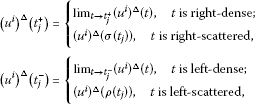$u\left(t\right)=\left({u}^{1}\left(t\right),{u}^{2}\left(t\right),\dots ,{u}^{N}\left(t\right)\right)$, $A\left(t\right)=\left[{d}_{lm}\left(t\right)\right]$ is a symmetric $N×N$ matrix-valued function defined on ${\left[0,T\right]}_{\mathbb{T}}$ with ${d}_{lm}\in {L}^{\mathrm{\infty }}\left({\left[0,T\right]}_{\mathbb{T}},\mathbb{R}\right)$ for all $l,m=1,2,\dots ,N$, ${I}_{ij}:\mathbb{R}\to \mathbb{R}$ ($i=1,2,\dots ,N,j=1,2,\dots ,p$) are continuous and $F:{\left[0,T\right]}_{\mathbb{T}}×{\mathbb{R}}^{N}\to \mathbb{R}$ satisfies the following assumption:

1. (A)

$F\left(t,x\right)$ is Δ-measurable in t for every $x\in {\mathbb{R}}^{N}$ and continuously differentiable in x for Δ-a.e. $t\in {\left[0,T\right]}_{\mathbb{T}}$, and there exist $a\in C\left({\mathbb{R}}^{+},{\mathbb{R}}^{+}\right)$, ${b}^{\sigma }\in {L}^{1}\left(0,T;{\mathbb{R}}^{+}\right)$ such that

$|F\left(t,x\right)|\le a\left(|x|\right)b\left(t\right),\phantom{\rule{2em}{0ex}}|\mathrm{\nabla }F\left(t,x\right)|\le a\left(|x|\right)b\left(t\right)$

for all $x\in {\mathbb{R}}^{N}$ and Δ-a.e. $t\in {\left[0,T\right]}_{\mathbb{T}}$, where $\mathrm{\nabla }F\left(t,x\right)$ denotes the gradient of $F\left(t,x\right)$ in x.

For the sake of convenience, in the sequel, we denote $\mathrm{\Gamma }=\left\{1,2,3,\dots ,N\right\}$, $\mathrm{\Lambda }=\left\{1,2,3,\dots ,p\right\}$.

When ${I}_{ij}\equiv 0$, $i\in A$, $j\in B$ and $A\left(t\right)$ is a zero matrix, (1.1) is the Hamiltonian system on time scales

(1.2)

In , the authors study the Sobolev’s spaces on time scales and their properties. As applications, they present a recent approach via variational methods and the critical point theory to obtain the existence of solutions for (1.2).

When ${I}_{ij}\left(t\right)\not\equiv 0$, $i\in A$, $j\in B$ and $A\left(t\right)$ is not a zero matrix, until now the variational structure of (1.1) has not been studied.

Problem (1.1) covers the second-order Hamiltonian system with impulsive effects (when $\mathbb{T}=\mathbb{R}$)

(1.3)

as well as the second-order discrete Hamiltonian system (when $\mathbb{T}=\mathbb{Z}$, $T\in \mathbb{N}$, $T\ge 2$)

$\left\{\begin{array}{c}u\left(t+2\right)-2u\left(t+1\right)+u\left(t\right)+A\left(t+1\right)u\left(t+1\right)\hfill \\ \phantom{\rule{1em}{0ex}}+\mathrm{\nabla }F\left(t+1,u\left(t+1\right)\right)=0,\phantom{\rule{1em}{0ex}}t\in \left[1,T-1\right]\cap \mathbb{Z},\hfill \\ u\left(0\right)-u\left(T\right)=0,u\left(T\right)-u\left(0\right)=u\left(T+1\right)-u\left(1\right),\hfill \\ {u}^{i}\left({t}_{j}+2\right)-{u}^{i}\left({t}_{j}+1\right)-{u}^{i}\left({t}_{j}\right)+{u}^{i}\left({t}_{j}-1\right)={I}_{ij}\left({u}^{i}\left({t}_{j}\right)\right),\phantom{\rule{1em}{0ex}}i\in \mathrm{\Gamma },j\in \mathrm{\Lambda }.\hfill \end{array}$

In , the authors establish some sufficient conditions on the existence of solutions of (1.3) by means of some critical point theorems when $A\left(t\right)\equiv 0$. When $\mathbb{T}\ne \mathbb{R}$, until now, it is unknown whether problem (1.1) has a variational structure or not.

Impulsive effects exist widely in many evolution processes in which their states are changed abruptly at certain moments of time. The theory of impulsive differential systems has been developed by numerous mathematicians (see ). Applications of impulsive differential equations with or without delays occur in biology, medicine, mechanics, engineering, chaos theory and so on (see ).

For a second-order differential equation ${u}^{″}=f\left(t,u,{u}^{\prime }\right)$, one usually considers impulses in the position u and the velocity ${u}^{\prime }$. However, in the motion of spacecraft, one has to consider instantaneous impulses depending on the position that result in jump discontinuities in velocity, but with no change in position (see ). The impulses only on the velocity occur also in impulsive mechanics (see ). An impulsive problem with impulses in the derivative only is considered in .

The study of dynamical systems on time scales is now an active area of research. One of the reasons for this is the fact that the study on time scales unifies the study of both discrete and continuous processes, besides many others. The pioneering works in this direction are Refs. . The theory of time scales was initiated by Stefan Hilger in his Ph.D. thesis in 1988, providing a rich theory that unifies and extends discrete and continuous analysis [18, 19]. The time scales calculus has a tremendous potential for applications in some mathematical models of real processes and phenomena studied in physics, chemical technology, population dynamics, biotechnology and economics, neural networks and social sciences (see ). For example, it can model insect populations that are continuous while in season (and may follow a difference scheme with variable step-size), die out in winter, while their eggs are incubating or dormant, and then hatch in a new season, giving rise to a nonoverlapping population.

There have been many approaches to study solutions of differential equations on time scales, such as the method of lower and upper solutions, fixed-point theory, coincidence degree theory and so on (see [1, 2029]). In , authors used the fixed point theorem of strict-set-contraction to study the existence of positive periodic solutions for functional differential equations with impulse effects on time scales. However, the study of the existence and multiplicity of solutions for differential equations on time scales using the variational method has received considerably less attention (see, for example, [1, 29]). The variational method is, to the best of our knowledge, novel and it may open a new approach to deal with nonlinear problems, with some type of discontinuities such as impulses.

Motivated by the above, we research the existence of variational construction for problem (1.1) in an appropriate space of functions and study the existence of solutions for (1.1) by some critical point theorems in this paper. All these results are new.

## 2 Preliminaries and statements

In this section, we present some fundamental definitions and results from the calculus on time scales and Sobolev’s spaces on time scales that will be required below. These are a generalization to ${R}^{n}$ of definitions and results found in .

Definition 2.1 ([, Definition 1.1])

Let $\mathbb{T}$ be a time scale. For $t\in \mathbb{T}$, the forward jump operator $\sigma :\mathbb{T}\to \mathbb{T}$ is defined by

while the backward jump operator $\rho :\mathbb{T}\to \mathbb{T}$ is defined by

(supplemented by $inf\mathrm{\varnothing }=sup\mathbb{T}$ and $sup\mathrm{\varnothing }=inf\mathbb{T}$, where denotes the empty set). A point $t\in \mathbb{T}$ is called right-scattered, left-scattered, if $\sigma \left(t\right)>t$, $\rho \left(t\right) hold, respectively. Points that are right-scattered and left-scattered at the same time are called isolated. Also, if $t and $\sigma \left(t\right)=t$, then t is called right-dense, and if $t>inf\mathbb{T}$ and $\rho \left(t\right)=t$, then t is called left-dense. Points that are right-dense and left-dense at the same time are called dense. The set ${\mathbb{T}}^{\kappa }$ which is derived from the time scale $\mathbb{T}$ as follows. If $\mathbb{T}$ has a left-scattered maximum m, then ${\mathbb{T}}^{\kappa }=\mathbb{T}-\left\{m\right\}$; otherwise, ${\mathbb{T}}^{\kappa }=\mathbb{T}$.

When $a,b\in \mathbb{T}$, $a, we denote the intervals ${\left[a,b\right]}_{\mathbb{T}}$, ${\left[a,b\right)}_{\mathbb{T}}$ and ${\left(a,b\right]}_{\mathbb{T}}$ in $\mathbb{T}$ by

${\left[a,b\right]}_{\mathbb{T}}=\left[a,b\right]\cap \mathbb{T},\phantom{\rule{2em}{0ex}}{\left[a,b\right)}_{\mathbb{T}}=\left[a,b\right)\cap \mathbb{T},\phantom{\rule{2em}{0ex}}{\left(a,b\right]}_{\mathbb{T}}=\left(a,b\right]\cap \mathbb{T},$

respectively. Note that ${\left[a,b\right]}_{\mathbb{T}}^{\kappa }={\left[a,b\right]}_{\mathbb{T}}$ if b is left-dense and ${\left[a,b\right]}_{\mathbb{T}}^{\kappa }={\left[a,b\right)}_{\mathbb{T}}={\left[a,\rho \left(b\right)\right]}_{\mathbb{T}}$ if b is left-scattered. We denote ${\left[a,b\right]}_{\mathbb{T}}^{{\kappa }^{2}}={\left({\left[a,b\right]}_{\mathbb{T}}^{\kappa }\right)}^{\kappa }$, therefore ${\left[a,b\right]}_{\mathbb{T}}^{{\kappa }^{2}}={\left[a,b\right]}_{\mathbb{T}}$ if b is left-dense and ${\left[a,b\right]}_{\mathbb{T}}^{{\kappa }^{2}}={\left[a,\rho \left(b\right)\right]}_{\mathbb{T}}^{\kappa }$ if b is left-scattered.

Definition 2.2 ([, Definition 1.10])

Assume that $f:\mathbb{T}\to \mathbb{R}$ is a function and let $t\in {\mathbb{T}}^{\kappa }$. Then we define ${f}^{\mathrm{\Delta }}\left(t\right)$ to be the number (provided it exists) with the property that given any $ϵ>0$, there is a neighborhood U of t (i.e., $U=\left(t-\delta ,t+\delta \right)\cap \mathbb{T}$ for some $\delta >0$) such that

We call ${f}^{\mathrm{\Delta }}\left(t\right)$ the delta (or Hilger) derivative of f at t. The function f is delta (or Hilger) differentiable on ${\mathbb{T}}^{\kappa }$ provided ${f}^{\mathrm{\Delta }}\left(t\right)$ exists for all $t\in {\mathbb{T}}^{\kappa }$. The function ${f}^{\mathrm{\Delta }}:{\mathbb{T}}^{\kappa }\to \mathbb{R}$ is then called the delta derivative of f on ${\mathbb{T}}^{\kappa }$.

Definition 2.3 ([, Definition 2.3])

Assume that $f:\mathbb{T}\to {\mathbb{R}}^{N}$ is a function,

$f\left(t\right)=\left({f}^{1}\left(t\right),{f}^{2}\left(t\right),\dots ,{f}^{N}\left(t\right)\right),$

and let $t\in {\mathbb{T}}^{\kappa }$. Then we define ${f}^{\mathrm{\Delta }}\left(t\right)=\left({f}^{{1}^{\mathrm{\Delta }}}\left(t\right),{f}^{{2}^{\mathrm{\Delta }}}\left(t\right),\dots ,{f}^{{N}^{\mathrm{\Delta }}}\left(t\right)\right)$ (provided it exists). We call ${f}^{\mathrm{\Delta }}\left(t\right)$ the delta (or Hilger) derivative of f at t. The function f is delta (or Hilger) differentiable provided ${f}^{\mathrm{\Delta }}\left(t\right)$ exists for all $t\in {\mathbb{T}}^{\kappa }$. The function ${f}^{\mathrm{\Delta }}:{\mathbb{T}}^{\kappa }\to {\mathbb{R}}^{N}$ is then called the delta derivative of f on ${\mathbb{T}}^{\kappa }$.

Definition 2.4 ([, Definition 2.7])

For a function $f:\mathbb{T}\to \mathbb{R}$, we will talk about the second derivative ${f}^{{\mathrm{\Delta }}^{2}}$ provided ${f}^{\mathrm{\Delta }}$ is differentiable on ${\mathbb{T}}^{{\kappa }^{2}}={\left({\mathbb{T}}^{\kappa }\right)}^{\kappa }$ with derivative ${f}^{{\mathrm{\Delta }}^{2}}={\left({f}^{\mathrm{\Delta }}\right)}^{\mathrm{\Delta }}:{\mathbb{T}}^{{\kappa }^{2}}\to \mathbb{R}$.

Definition 2.5 ([, Definition 2.5])

For a function $f:\mathbb{T}\to {\mathbb{R}}^{N}$, we will talk about the second derivative ${f}^{{\mathrm{\Delta }}^{2}}$ provided ${f}^{\mathrm{\Delta }}$ is differentiable on ${\mathbb{T}}^{{\kappa }^{2}}={\left({\mathbb{T}}^{\kappa }\right)}^{\kappa }$ with derivative ${f}^{{\mathrm{\Delta }}^{2}}={\left({f}^{\mathrm{\Delta }}\right)}^{\mathrm{\Delta }}:{\mathbb{T}}^{{\kappa }^{2}}\to {\mathbb{R}}^{N}$.

The Δ-measure ${\mu }_{\mathrm{\Delta }}$ and Δ-integration are defined as those in .

Definition 2.6 ([, Definition 2.7])

Assume that $f:\mathbb{T}\to {\mathbb{R}}^{N}$ is a function, $f\left(t\right)=\left({f}^{1}\left(t\right),{f}^{2}\left(t\right),\dots ,{f}^{N}\left(t\right)\right)$ and let A be a Δ-measurable subset of $\mathbb{T}$. f is integrable on A if and only if ${f}^{i}$ ($i=1,2,\dots ,N$) are integrable on A, and ${\int }_{A}f\left(t\right)\mathrm{\Delta }t=\left({\int }_{A}{f}^{1}\left(t\right)\mathrm{\Delta }t,{\int }_{A}{f}^{2}\left(t\right)\mathrm{\Delta }t,\dots ,{\int }_{A}{f}^{N}\left(t\right)\mathrm{\Delta }t\right)$.

Definition 2.7 ([, Definition 2.3])

Let $B\subset \mathbb{T}$. B is called a Δ-null set if ${\mu }_{\mathrm{\Delta }}\left(B\right)=0$. Say that a property P holds Δ-almost everywhere (Δ-a.e.) on B, or for Δ-almost all (Δ-a.a.) $t\in B$ if there is a Δ-null set ${E}_{0}\subset B$ such that P holds for all $t\in B\mathrm{\setminus }{E}_{0}$.

For $p\in \mathbb{R}$, $p\ge 1$, we set the space

${L}_{\mathrm{\Delta }}^{p}\left({\left[0,T\right)}_{\mathbb{T}},{\mathbb{R}}^{N}\right)=\left\{u:{\left[0,T\right)}_{\mathbb{T}}\to {\mathbb{R}}^{N}:{\int }_{{\left[0,T\right)}_{\mathbb{T}}}{|f\left(t\right)|}^{p}\mathrm{\Delta }t<+\mathrm{\infty }\right\}$

with the norm

${\parallel f\parallel }_{{L}_{\mathrm{\Delta }}^{p}}={\left({\int }_{{\left[0,T\right)}_{\mathbb{T}}}{|f\left(t\right)|}^{p}\mathrm{\Delta }t\right)}^{\frac{1}{p}}.$

We have the following theorem.

Theorem 2.1 ([, Theorem 2.1])

Let $p\in \mathbb{R}$ be such that $p\ge 1$. Then the space ${L}_{\mathrm{\Delta }}^{p}\left({\left[0,T\right)}_{\mathbb{T}},{\mathbb{R}}^{N}\right)$ is a Banach space together with the norm ${\parallel \cdot \parallel }_{{L}_{\mathrm{\Delta }}^{p}}$. Moreover, ${L}_{\mathrm{\Delta }}^{2}\left({\left[a,b\right)}_{\mathbb{T}},{\mathbb{R}}^{N}\right)$ is a Hilbert space together with the inner product given for every $\left(f,g\right)\in {L}_{\mathrm{\Delta }}^{p}\left({\left[a,b\right)}_{\mathbb{T}},{\mathbb{R}}^{N}\right)×{L}_{\mathrm{\Delta }}^{p}\left({\left[a,b\right)}_{\mathbb{T}},{\mathbb{R}}^{N}\right)$ by

${〈f,g〉}_{{L}_{\mathrm{\Delta }}^{2}}={\int }_{{\left[a,b\right)}_{\mathbb{T}}}\left(f\left(t\right),g\left(t\right)\right)\mathrm{\Delta }t,$

where $\left(\cdot ,\cdot \right)$ denotes the inner product in ${\mathbb{R}}^{N}$.

Definition 2.8 ([, Definition 2.11])

A function $f:{\left[a,b\right]}_{\mathbb{T}}\to {\mathbb{R}}^{N},f\left(t\right)=\left({f}^{1}\left(t\right),{f}^{2}\left(t\right),\dots ,{f}^{N}\left(t\right)\right)$. We say that f is absolutely continuous on ${\left[a,b\right]}_{\mathbb{T}}$ (i.e., $f\in AC\left({\left[a,b\right]}_{\mathbb{T}},{\mathbb{R}}^{N}\right)$) if for every $ϵ>0$, there exists $\delta >0$ such that if ${\left\{{\left[{a}_{k},{b}_{k}\right)}_{\mathbb{T}}\right\}}_{k=1}^{n}$ is a finite pairwise disjoint family of subintervals of ${\left[a,b\right]}_{\mathbb{T}}$ satisfying ${\sum }_{k=1}^{n}\left({b}_{k}-{a}_{k}\right)<\delta$, then ${\sum }_{k=1}^{n}|f\left({b}_{k}\right)-f\left({a}_{k}\right)|<ϵ$.

Now, we recall the Sobolev space ${W}_{\mathrm{\Delta },T}^{1,p}\left({\left[0,T\right]}_{\mathbb{T}},{\mathbb{R}}^{N}\right)$ on ${\left[0,T\right]}_{\mathbb{T}}$ defined in . For the sake of convenience, in the sequel we let ${u}^{\sigma }\left(t\right)=u\left(\sigma \left(t\right)\right)$.

Definition 2.9 ([, Definition 2.12])

Let $p\in \mathbb{R}$ be such that $p>1$ and $u:{\left[0,T\right]}_{\mathbb{T}}\to {\mathbb{R}}^{N}$. We say that $u\in {W}_{\mathrm{\Delta },T}^{1,p}\left({\left[0,T\right]}_{\mathbb{T}},{\mathbb{R}}^{N}\right)$ if and only if $u\in {L}_{\mathrm{\Delta }}^{p}\left({\left[0,T\right)}_{\mathbb{T}},{\mathbb{R}}^{N}\right)$ and there exists $g:{\left[0,T\right]}_{\mathbb{T}}^{\kappa }\to {\mathbb{R}}^{N}$ such $g\in {L}_{\mathrm{\Delta }}^{p}\left({\left[0,T\right)}_{\mathbb{T}},{\mathbb{R}}^{N}\right)$ and

${\int }_{{\left[0,T\right)}_{\mathbb{T}}}\left(u\left(t\right),{\varphi }^{\mathrm{\Delta }}\left(t\right)\right)\mathrm{\Delta }t=-{\int }_{{\left[0,T\right)}_{\mathbb{T}}}\left(g\left(t\right),{\varphi }^{\sigma }\left(t\right)\right)\mathrm{\Delta }t,\phantom{\rule{1em}{0ex}}\mathrm{\forall }\varphi \in {C}_{T,rd}^{1}\left({\left[0,T\right]}_{\mathbb{T}},{\mathbb{R}}^{N}\right).$
(2.1)

For $p\in \mathbb{R}$, $p>1$, we denote

${V}_{\mathrm{\Delta },T}^{1,p}\left({\left[0,T\right]}_{\mathbb{T}},{\mathbb{R}}^{N}\right)=\left\{x\in AC\left({\left[0,T\right]}_{\mathbb{T}},{\mathbb{R}}^{N}\right):{x}^{\mathrm{\Delta }}\in {L}_{\mathrm{\Delta }}^{p}\left({\left[0,T\right)}_{\mathbb{T}},{\mathbb{R}}^{N}\right),x\left(0\right)=x\left(T\right)\right\}.$

It follows from Remark 2.2 in  that

${V}_{\mathrm{\Delta }}^{1,p}\left({\left[0,T\right]}_{\mathbb{T}},{\mathbb{R}}_{N}\right)\subset {W}_{\mathrm{\Delta }}^{1,p}\left({\left[0,T\right]}_{\mathbb{T}},{\mathbb{R}}^{N}\right)$

is true for every $p\in \mathbb{R}$ with $p>1$. These two sets are, as a class of functions, equivalent. It is the characterization of functions in ${W}_{\mathrm{\Delta },T}^{1,p}\left({\left[0,T\right]}_{\mathbb{T}},{\mathbb{R}}^{N}\right)$ in terms of functions in ${V}_{\mathrm{\Delta },T}^{1,p}\left({\left[0,T\right]}_{\mathbb{T}},{\mathbb{R}}^{N}\right)$ too. That is the following theorem.

Theorem 2.2 ([, Theorem 2.5])

Suppose that $u\in {W}_{\mathrm{\Delta },T}^{1,p}\left({\left[0,T\right]}_{\mathbb{T}},{\mathbb{R}}^{N}\right)$ for some $p\in \mathbb{R}$ with $p>1$, and that (2.1) holds for $g\in {L}_{\mathrm{\Delta }}^{p}\left({\left[0,T\right)}_{\mathbb{T}},{\mathbb{R}}^{N}\right)$. Then there exists a unique function $x\in {V}_{\mathrm{\Delta },T}^{1,p}\left({\left[0,T\right]}_{\mathbb{T}},{\mathbb{R}}^{N}\right)$ such that the equalities

$x=u,\phantom{\rule{2em}{0ex}}{x}^{\mathrm{\Delta }}=g\phantom{\rule{1em}{0ex}}\mathrm{\Delta }\mathit{\text{-a.e. on}}\phantom{\rule{0.5em}{0ex}}{\left[0,T\right)}_{\mathbb{T}}$
(2.2)

are satisfied and

${\int }_{{\left[0,T\right)}_{\mathbb{T}}}g\left(t\right)\mathrm{\Delta }t=0.$
(2.3)

By identifying $u\in {W}_{\mathrm{\Delta },T}^{1,p}\left({\left[0,T\right]}_{\mathbb{T}},{\mathbb{R}}^{N}\right)$ with its absolutely continuous representative $x\in {V}_{\mathrm{\Delta },T}^{1,p}\left({\left[0,T\right]}_{\mathbb{T}},{\mathbb{R}}^{N}\right)$ for which (2.2) holds, the set ${W}_{\mathrm{\Delta },T}^{1,p}\left({\left[0,T\right]}_{\mathbb{T}},{\mathbb{R}}^{N}\right)$ can be endowed with the structure of a Banach space. That is the following theorem.

Theorem 2.3 ([, Theorem 2.21])

Assume $p\in \mathbb{R}$ and $p>1$. The set ${W}_{\mathrm{\Delta },T}^{1,p}\left({\left[0,T\right]}_{\mathbb{T}},{\mathbb{R}}^{N}\right)$ is a Banach space together with the norm defined as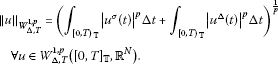(2.4)

Moreover, the set ${H}_{\mathrm{\Delta },T}^{1}={W}_{\mathrm{\Delta },T}^{1,2}\left({\left[0,T\right]}_{\mathbb{T}},{\mathbb{R}}^{N}\right)$ is a Hilbert space together with the inner product

${〈u,v〉}_{{H}_{\mathrm{\Delta },T}^{1}}={\int }_{{\left[0,T\right)}_{\mathbb{T}}}\left({u}^{\sigma }\left(t\right),{v}^{\sigma }\left(t\right)\right)\mathrm{\Delta }t+{\int }_{{\left[0,T\right)}_{\mathbb{T}}}\left({u}^{\mathrm{\Delta }}\left(t\right),{v}^{\mathrm{\Delta }}\left(t\right)\right)\mathrm{\Delta }t\phantom{\rule{1em}{0ex}}\mathrm{\forall }u,v\in {H}_{\mathrm{\Delta },T}^{1}.$

The Banach space ${W}_{\mathrm{\Delta },T}^{1,p}\left({\left[0,T\right]}_{\mathbb{T}},{\mathbb{R}}^{N}\right)$ has some important properties.

Theorem 2.4 ([, Theorem 2.23])

There exists ${C}_{1}>0$ such that the inequality

${\parallel u\parallel }_{\mathrm{\infty }}\le {C}_{1}{\parallel u\parallel }_{{H}_{\mathrm{\Delta },T}^{1}}$
(2.5)

holds for all $u\in {H}_{\mathrm{\Delta },T}^{1}$, where ${\parallel u\parallel }_{\mathrm{\infty }}={max}_{t\in {\left[0,T\right]}_{\mathbb{T}}}|u\left(t\right)|$.

Moreover, if ${\int }_{{\left[0,T\right)}_{\mathbb{T}}}u\left(t\right)\mathrm{\Delta }t=0$, then

${\parallel u\parallel }_{\mathrm{\infty }}\le {C}_{1}{\parallel {u}^{\mathrm{\Delta }}\parallel }_{{L}_{\mathrm{\Delta }}^{2}}.$

Theorem 2.5 ([, Theorem 2.25])

If the sequence ${\left\{{u}_{k}\right\}}_{k\in \mathbb{N}}\subset {W}_{\mathrm{\Delta },T}^{1,p}\left({\left[0,T\right]}_{\mathbb{T}},{\mathbb{R}}^{N}\right)$ converges weakly to u in ${W}_{\mathrm{\Delta },T}^{1,p}\left({\left[0,T\right]}_{\mathbb{T}},{\mathbb{R}}^{N}\right)$, then ${\left\{{u}_{k}\right\}}_{k\in \mathbb{N}}$ converges strongly in $C\left({\left[0,T\right]}_{\mathbb{T}},{\mathbb{R}}^{N}\right)$ to u.

Theorem 2.6 ([, Theorem 2.27])

Let $L:{\left[0,T\right]}_{\mathbb{T}}×{\mathbb{R}}^{N}×{\mathbb{R}}^{N}\to \mathbb{R},\left(t,x,y\right)\to L\left(t,x,y\right)$ be Δ-measurable in t for each $\left(x,y\right)\in {\mathbb{R}}^{N}×{\mathbb{R}}^{N}$ and continuously differentiable in $\left(x,y\right)$ for Δ-almost every $t\in {\left[0,T\right]}_{\mathbb{T}}$. If there exist $a\in C\left({\mathbb{R}}^{+},{\mathbb{R}}^{+}\right)$, $b\in {L}_{\mathrm{\Delta }}^{1}\left({\left[0,T\right]}_{\mathbb{T}},{\mathbb{R}}^{+}\right)$ and $c\in {L}_{\mathrm{\Delta }}^{q}\left({\left[0,T\right]}_{\mathbb{T}},{\mathbb{R}}^{+}\right)$ ($1) such that for Δ-almost $t\in {\left[0,T\right]}_{\mathbb{T}}$ and every $\left(x,y\right)\in {\mathbb{R}}^{N}×{\mathbb{R}}^{N}$, one has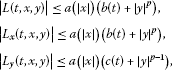(2.6)

where $\frac{1}{p}+\frac{1}{q}=1$, then the functional $\mathrm{\Phi }:{W}_{\mathrm{\Delta },T}^{1,p}\left({\left[0,T\right]}_{\mathbb{T}},{\mathbb{R}}^{N}\right)\to \mathbb{R}$ defined as

$\mathrm{\Phi }\left(u\right)={\int }_{{\left[0,T\right)}_{\mathbb{T}}}L\left(\sigma \left(t\right),{u}^{\sigma }\left(t\right),{u}^{\mathrm{\Delta }}\left(t\right)\right)\mathrm{\Delta }t$

is continuously differentiable on ${W}_{\mathrm{\Delta },T}^{1,p}\left({\left[0,T\right]}_{\mathbb{T}},{\mathbb{R}}^{N}\right)$ and

$\begin{array}{rcl}〈{\mathrm{\Phi }}^{\prime }\left(u\right),v〉& =& {\int }_{{\left[0,T\right)}_{\mathbb{T}}}\left[\left({L}_{x}\left(\sigma \left(t\right),{u}^{\sigma }\left(t\right),{u}^{\mathrm{\Delta }}\left(t\right)\right),{v}^{\sigma }\left(t\right)\right)\\ +\left({L}_{y}\left(\sigma \left(t\right),{u}^{\sigma }\left(t\right),{u}^{\mathrm{\Delta }}\left(t\right)\right),{v}^{\mathrm{\Delta }}\left(t\right)\right)\right]\mathrm{\Delta }t.\end{array}$
(2.7)

## 3 Variational setting

In this section, we recall some basic facts which will be used in the proofs of our main results. In order to apply the critical point theory, we make a variational structure. From this variational structure, we can reduce the problem of finding solutions of (1.1) to the one of seeking the critical points of a corresponding functional.

If $u\in {H}_{\mathrm{\Delta },T}^{1}$, by identifying $u\in {H}_{\mathrm{\Delta },T}^{1}$ with its absolutely continuous representative $x\in {V}_{\mathrm{\Delta },T}^{1,2}\left({\left[0,T\right]}_{\mathbb{T}},{\mathbb{R}}^{N}\right)$ for which (2.2) holds, then u is absolutely continuous and $\stackrel{˙}{u}\in {L}^{2}\left({\left[0,T\right)}_{\mathbb{T}};{\mathbb{R}}^{N}\right)$. In this case, ${u}^{\mathrm{\Delta }}\left({t}^{+}\right)-{u}^{\mathrm{\Delta }}\left({t}^{-}\right)=0$ may not hold for some $t\in {\left(0,T\right)}_{\mathbb{T}}$. This leads to impulsive effects.

Take $v\in {H}_{\mathrm{\Delta },T}^{1}$ and multiply the two sides of the equality

${u}^{{\mathrm{\Delta }}^{2}}\left(t\right)+A\left(\sigma \left(t\right)\right)u\left(\sigma \left(t\right)\right)+\mathrm{\nabla }F\left(\sigma \left(t\right),{u}^{\sigma }\left(t\right)\right)=0$

by ${v}^{\sigma }$ and integrate on ${\left[0,T\right)}_{\mathbb{T}}$, then we have

${\int }_{{\left[0,T\right)}_{\mathbb{T}}}\left[{u}^{{\mathrm{\Delta }}^{2}}\left(t\right)+A\left(\sigma \left(t\right)\right)u\left(\sigma \left(t\right)\right)+\mathrm{\nabla }F\left(\sigma \left(t\right),{u}^{\sigma }\left(t\right)\right)\right]{v}^{\sigma }\left(t\right)\mathrm{\Delta }t=0.$
(3.1)

Moreover, combining ${u}^{\mathrm{\Delta }}\left(0\right)-{u}^{\mathrm{\Delta }}\left(T\right)=0$, one has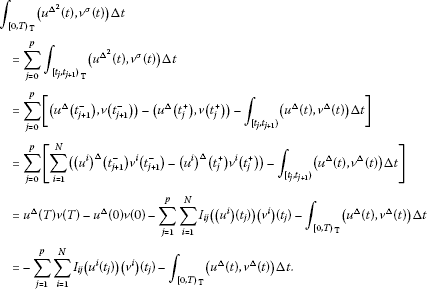Combining (3.1), we have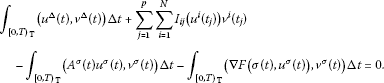Considering the above, we introduce the following concept solution for problem (1.1).

Definition 3.1 We say that a function $u\in {H}_{\mathrm{\Delta },T}^{1}$ is a weak solution of problem (1.1) if the identity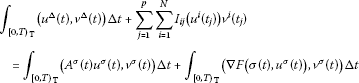holds for any $v\in {H}_{\mathrm{\Delta },T}^{1}$.

Consider the functional $\phi :{H}_{\mathrm{\Delta },T}^{1}\to \mathbb{R}$ defined by

$\begin{array}{rcl}\phi \left(u\right)& =& \frac{1}{2}{\int }_{{\left[0,T\right)}_{\mathbb{T}}}{|{u}^{\mathrm{\Delta }}\left(t\right)|}^{2}\mathrm{\Delta }t+\sum _{j=1}^{p}\sum _{i=1}^{N}{\int }_{0}^{{u}^{i}\left({t}_{j}\right)}{I}_{ij}\left(t\right)\phantom{\rule{0.2em}{0ex}}\mathrm{d}t\\ -\frac{1}{2}{\int }_{{\left[0,T\right)}_{\mathbb{T}}}\left({A}^{\sigma }\left(t\right){u}^{\sigma }\left(t\right),{u}^{\sigma }\left(t\right)\right)\mathrm{\Delta }t+J\left(u\right)\\ =& \psi \left(u\right)+\varphi \left(u\right),\end{array}$
(3.2)

where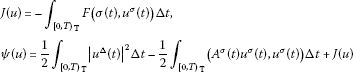and

$\varphi \left(u\right)=\sum _{j=1}^{p}\sum _{i=1}^{N}{\int }_{0}^{{u}^{i}\left({t}_{j}\right)}{I}_{ij}\left(t\right)\phantom{\rule{0.2em}{0ex}}\mathrm{d}t.$

Lemma 3.1 The functional φ is continuously differentiable on ${H}_{\mathrm{\Delta },T}^{1}$ and

$\begin{array}{rcl}〈{\phi }^{\prime }\left(u\right),v〉& =& {\int }_{{\left[0,T\right)}_{\mathbb{T}}}\left({u}^{\mathrm{\Delta }}\left(t\right),{v}^{\mathrm{\Delta }}\left(t\right)\right)\mathrm{\Delta }t+\sum _{j=1}^{p}\sum _{i=1}^{N}{I}_{ij}\left({u}^{i}\left({t}_{j}\right)\right){v}^{i}\left({t}_{j}\right)\\ -{\int }_{{\left[0,T\right)}_{\mathbb{T}}}\left[\left({A}^{\sigma }\left(t\right){u}^{\sigma }\left(t\right),{v}^{\sigma }\left(t\right)\right)-\left(\mathrm{\nabla }F\left(\sigma \left(t\right),{u}^{\sigma }\left(t\right)\right),{v}^{\sigma }\left(t\right)\right)\right]\mathrm{\Delta }t.\end{array}$
(3.3)

Proof Set $L\left(t,x,y\right)=\frac{1}{2}{|y|}^{2}-\frac{1}{2}\left(A\left(t\right)x,x\right)-F\left(t,x\right)$ for all $x,y\in {R}^{N}$ and $t\in {\left[0,T\right]}_{\mathbb{T}}$. Then $L\left(t,x,y\right)$ satisfies all assumptions of Theorem 2.6. Hence, by Theorem 2.6, we know that the functional ψ is continuously differentiable on ${H}_{\mathrm{\Delta },T}^{1}$ and

$〈{\psi }^{\prime }\left(u\right),v〉={\int }_{{\left[0,T\right)}_{\mathbb{T}}}\left[\left({u}^{\mathrm{\Delta }}\left(t\right),{v}^{\mathrm{\Delta }}\left(t\right)\right)-\left({A}^{\sigma }\left(t\right){u}^{\sigma }\left(t\right),{v}^{\sigma }\left(t\right)\right)-\left(\mathrm{\nabla }F\left(\sigma \left(t\right),{u}^{\sigma }\left(t\right)\right),{v}^{\sigma }\left(t\right)\right)\right]\mathrm{\Delta }t$

for all $u,v\in {H}_{\mathrm{\Delta },T}^{1}$.

On the other hand, by the continuity of ${I}_{ij}$, $i\in \mathrm{\Gamma }$, $j\in \mathrm{\Lambda }$, one has that $\varphi \in {C}^{1}\left({H}_{\mathrm{\Delta },T}^{1},\mathbb{R}\right)$ and

$〈{\varphi }^{\prime }\left(u\right),v〉=\sum _{j=1}^{p}\sum _{i=1}^{N}{I}_{ij}\left({u}^{i}\left({t}_{j}\right)\right){v}^{i}\left({t}_{j}\right)$

for all $u,v\in {H}_{\mathrm{\Delta },T}^{1}$. Thus, φ is continuously differentiable on ${H}_{\mathrm{\Delta },T}^{1}$ and (3.3) holds. □

By Definition 3.1 and Lemma 3.1, the weak solutions of problem (1.1) correspond to the critical points of φ.

Moreover, we need more preliminaries. For any $u\in {H}_{\mathrm{\Delta },T}^{1}$, let

$q\left(u\right)=\frac{1}{2}{\int }_{{\left[0,T\right)}_{\mathbb{T}}}\left[{|{u}^{\sigma }\left(t\right)|}^{2}-\left({A}^{\sigma }\left(t\right){u}^{\sigma }\left(t\right),{u}^{\sigma }\left(t\right)\right)\right]\mathrm{\Delta }t.$

We see that

$\begin{array}{rcl}q\left(u\right)& =& \frac{1}{2}{\parallel u\parallel }^{2}-\frac{1}{2}{\int }_{{\left[0,T\right)}_{\mathbb{T}}}\left(\left({A}^{\sigma }\left(t\right)+{I}_{N×N}\right){u}^{\sigma }\left(t\right),{u}^{\sigma }\left(t\right)\right)\mathrm{\Delta }t\\ =& \frac{1}{2}〈\left(I-K\right)u,u〉,\end{array}$

where $K:{H}_{\mathrm{\Delta },T}^{1}\to {H}_{\mathrm{\Delta },T}^{1}$ is the bounded self-adjoint linear operator defined, using the Riesz representation theorem, by

$〈Ku,v〉={\int }_{{\left[0,T\right)}_{\mathbb{T}}}\left(\left({A}^{\sigma }\left(t\right)+{I}_{N×N}\right){u}^{\sigma }\left(t\right),{v}^{\sigma }\left(t\right)\right)\mathrm{\Delta }t,\phantom{\rule{1em}{0ex}}\mathrm{\forall }u,v\in {H}_{\mathrm{\Delta },T}^{1},$

${I}_{N×N}$ and I denote an $N×N$ identity matrix and an identity operator, respectively. By (3.2), $\phi \left(u\right)$ can be rewritten as

$\begin{array}{rcl}\phi \left(u\right)& =& q\left(u\right)+\varphi \left(u\right)+J\left(u\right)\\ =& \frac{1}{2}〈\left(I-K\right)u,u〉+\varphi \left(u\right)+J\left(u\right).\end{array}$
(3.4)

The compact imbedding of ${H}_{\mathrm{\Delta },T}^{1}$ into $C\left({\left[0,T\right]}_{\mathbb{T}},{R}^{N}\right)$ implies that K is compact. By classical spectral theory, we can decompose ${H}_{\mathrm{\Delta },T}^{1}$ into the orthogonal sum of invariant subspaces for $I-K$

${H}_{\mathrm{\Delta },T}^{1}={H}^{-}\oplus {H}^{0}\oplus {H}^{+},$

where ${H}^{0}=ker\left(I-K\right)$ and ${H}^{-}$, ${H}^{+}$ are such that, for some $\delta >0$,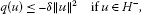(3.5)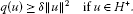(3.6)

Remark 3.1 K has only finitely many eigenvalues ${\lambda }_{i}$ with ${\lambda }_{i}>1$ since K is compact on ${H}_{T}^{1}$. Hence ${H}^{-}$ is finite dimensional. Notice that $I-K$ is a compact perturbation of the self-adjoint operator I. By a well-known theorem, we know that 0 is not in the essential spectrum of $I-K$. Hence, ${H}^{0}$ is a finite dimensional space too.

To prove our main results, we need the following definitions and theorems.

Definition 3.2 ([, ${P}_{81}$])

Let X be a real Banach space and $I\in {C}^{1}\left(X,\mathbb{R}\right)$. I is said to be satisfying (PS) condition on X if any sequence $\left\{{x}_{n}\right\}\subseteq X$ for which $I\left({x}_{n}\right)$ is bounded and ${I}^{\prime }\left({x}_{n}\right)\to 0$ as $n\to \mathrm{\infty }$, possesses a convergent subsequence in X.

Firstly, we state the local linking theorem.

Let X be a real Banach space with a direct decomposition $X={X}^{1}\oplus {X}^{2}$. Consider two sequences of a subspace

${X}_{0}^{1}\subset {X}_{1}^{1}\subset \cdots \subset {X}^{1},\phantom{\rule{2em}{0ex}}{X}_{0}^{2}\subset {X}_{1}^{2}\subset \cdots \subset {X}^{2}$

such that

$dim{X}_{n}^{1}<+\mathrm{\infty },\phantom{\rule{2em}{0ex}}dim{X}_{n}^{2}<+\mathrm{\infty },\phantom{\rule{1em}{0ex}}n\in \mathbb{N}$

and

${X}^{1}=\overline{\bigcup _{n\in \mathbb{N}}{X}_{n}^{1}},\phantom{\rule{2em}{0ex}}{X}^{2}=\overline{\bigcup _{n\in \mathbb{N}}{X}_{n}^{2}}.$

For every multi-index $\alpha =\left({\alpha }_{1},{\alpha }_{2}\right)\in {\mathbb{N}}^{2}$, we denote by ${X}_{\alpha }$ the space ${X}_{{\alpha }_{1}}\oplus {X}_{{\alpha }_{2}}$. We say $\alpha \le \beta ⇔{\alpha }_{1}\le {\beta }_{1}$, ${\alpha }_{2}\le {\beta }_{2}$. A sequence $\left({\alpha }_{n}\right)\subset {\mathbb{N}}^{2}$ is admissible if, for every $\alpha \in {\mathbb{N}}^{2}$, there is ${m}_{0}\in \mathbb{N}$ such that $n\ge {m}_{0}⇒{\alpha }_{n}\ge \alpha$.

Definition 3.3 ([, Definition 2.2])

Let $I\in {C}^{1}\left(X,\mathbb{R}\right)$. The functional I satisfies the ${\left(C\right)}^{\ast }$ condition if every sequence $\left({u}_{{\alpha }_{n}}\right)$ such that ${\alpha }_{n}$ is admissible and

${u}_{{\alpha }_{n}}\in {X}_{{\alpha }_{n}},sup|I\left({u}_{{\alpha }_{n}}\right)|<\mathrm{\infty },\phantom{\rule{1em}{0ex}}\left(1+\parallel {u}_{{\alpha }_{n}}\parallel \right){I}^{\prime }\left({u}_{{\alpha }_{n}}\right)\to 0$

contains a subsequence which converges to a critical point of I.

Theorem 3.1 [, Theorem 2.2]

Suppose that $I\in {C}^{1}\left(X,\mathbb{R}\right)$ satisfies the following assumptions:

(I1) ${X}^{1}\ne \left\{0\right\}$ and I has a local linking at 0 with respect to $\left({X}^{1},{X}^{2}\right)$; that is, for some $M>0$,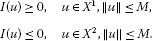(I2) I satisfies ${\left(C\right)}^{\ast }$ condition.

(I3) I maps bounded sets into bounded sets.

(I4) For every $n\in \mathbb{N}$, $I\left(u\right)\to -\mathrm{\infty }$ as $\parallel u\parallel \to \mathrm{\infty }$, $u\in {X}_{n}^{1}\oplus {X}^{2}$.

Then I has at least two critical points.

Remark 3.2 Since $I\in {C}^{1}\left(X,\mathbb{R}\right)$, by the condition (I1) of Theorem 3.1, 0 is the critical point of I. Thus, under the conditions of Theorem 3.1, I has at least one nontrivial critical point.

Secondly, we state another three critical point theorems.

Theorem 3.2 ([, Theorem 5.29])

Let E be a Hilbert space with $E={E}_{1}\oplus {E}_{2}$ and ${E}_{2}={E}_{1}^{\mathrm{\perp }}$. Suppose $I\in {C}^{1}\left(E,R\right)$, satisfies (PS) condition, and

(I5) $I\left(u\right)=\frac{1}{2}〈Lu,u〉+b\left(u\right)$, where $Lu={L}_{1}{P}_{1}u+{L}_{2}{P}_{2}u$ and ${L}_{\kappa }:{E}_{\kappa }\to {E}_{\kappa }$ is bounded and self-adjoint, $\kappa =1,2$,

(I6) ${b}^{\mathrm{\prime }}$ is compact, and

(I7) there exist a subspace $\stackrel{˜}{E}\subset E$ and sets $S\subset E$, $Q\subset \stackrel{˜}{E}$ and constants $\alpha >\omega$ such that

1. (i)

$S\subset {E}_{1}$ and $I{|}_{S}\ge \alpha$,

2. (ii)

Q is bounded and $I{|}_{\partial Q}\le \omega$,

3. (iii)

Then I possesses a critical value $c\ge \alpha$.

Theorem 3.3 ([, Theorem 9.12])

Let E be a Banach space. Let $I\in {C}^{1}\left(E,\mathbb{R}\right)$ be an even functional which satisfies the (PS) condition and $I\left(0\right)=0$. If $E=V\oplus W$, where V is finite dimensional, and I satisfies

(I8) there are constants $\rho ,\alpha >0$ such that $I{|}_{\partial {B}_{\rho }\cap W}\ge \alpha$, where ${B}_{\rho }=\left\{x\in E:\parallel x\parallel <\rho \right\}$,

(I9) for each finite dimensional subspace $\stackrel{˜}{E}\subset E$, there is an $R=R\left(\stackrel{˜}{E}\right)$ such that $I\le 0$ on $\stackrel{˜}{E}\mathrm{\setminus }{B}_{R\left(\stackrel{˜}{E}\right)}$,

then I possesses an unbounded sequence of critical values.

In order to state another critical point theorem, we need the following notions. Let X and Y be Banach spaces with X being separable and reflexive, and set $E=X\oplus Y$. Let $\mathcal{S}\subset {X}^{\ast }$ be a dense subset. For each $s\in \mathcal{S}$, there is a semi-norm on E defined by

We denote by ${\mathcal{T}}_{\mathcal{S}}$ the topology on E induced by a semi-norm family $\left\{{p}_{s}\right\}$, and let w and ${w}^{\ast }$ denote the weak-topology and weak*-topology, respectively.

For a functional $\mathrm{\Phi }\in {C}^{1}\left(E,R\right)$, we write ${\mathrm{\Phi }}_{a}=\left\{u\in E:\mathrm{\Phi }\left(u\right)\ge a\right\}$. Recall that ${\mathrm{\Phi }}^{\prime }$ is said to be weak sequentially continuous if, for any ${u}_{k}⇀u$ in E, one has ${lim}_{k\to \mathrm{\infty }}{\mathrm{\Phi }}^{\prime }\left({u}_{k}\right)v\to {\mathrm{\Phi }}^{\prime }\left(u\right)v$ for each $v\in E$, i.e., ${\mathrm{\Phi }}^{\prime }:\left(E,w\right)\to \left({E}^{\ast },{w}^{\ast }\right)$ is sequentially continuous. For $c\in R$, we say that Φ satisfies the ${\left(C\right)}_{c}$ condition if any sequence $\left\{{u}_{k}\right\}\subset E$ such that $\mathrm{\Phi }\left({u}_{k}\right)\to c$ and $\left(1+\parallel {u}_{k}\parallel \right){\mathrm{\Phi }}^{\prime }\left({u}_{k}\right)\to 0$ as $k\to \mathrm{\infty }$ contains a convergent subsequence.

Suppose that

(${\mathrm{\Phi }}_{0}$) for any $c\in R$, ${\mathrm{\Phi }}_{c}$ is ${\mathcal{T}}_{\mathcal{S}}$-closed, and ${\mathrm{\Phi }}^{\prime }:\left({\mathrm{\Phi }}_{c},{\mathcal{T}}_{\mathcal{S}}\right)\to \left({E}^{\ast },{w}^{\ast }\right)$ is continuous;

(${\mathrm{\Phi }}_{1}$) there exists $\rho >0$ such that $\kappa :=inf\mathrm{\Phi }\left(\partial {B}_{\rho }\cap Y\right)>0$, where

${B}_{\rho }=\left\{u\in E:\parallel u\parallel <\rho \right\};$

(${\mathrm{\Phi }}_{2}$) there exist a finite dimensional subspace ${Y}_{0}\subset Y$ and $R>\rho$ such that $\overline{c}:=sup\mathrm{\Phi }\left({E}_{0}\right)<\mathrm{\infty }$ and $sup\mathrm{\Phi }\left({E}_{0}\mathrm{\setminus }{S}_{0}\right), where

${E}_{0}:=X\oplus {Y}_{0},\phantom{\rule{1em}{0ex}}\text{and}\phantom{\rule{1em}{0ex}}{S}_{0}=\left\{u\in {E}_{0}:\parallel u\parallel \le R\right\}.$

Theorem 3.4 ()

Assume that Φ is even and (${\mathrm{\Phi }}_{0}$)-(${\mathrm{\Phi }}_{2}$) are satisfied. Then Φ has at least $m=dim{Y}_{0}$ pairs of critical points with critical values less than or equal to $\overline{c}$ provided Φ satisfies the ${\left(C\right)}_{c}$ condition for all $c\in \left[\kappa ,\overline{c}\right]$.

Remark 3.3 In our applications, we take $\mathcal{S}$=${X}^{\ast }$ so that ${\mathcal{T}}_{\mathcal{S}}$ is the product topology on $E=X\oplus Y$ given by the weak topology on X and the strong topology on Y.

## 4 Main results

Lemma 4.1 ${\varphi }^{\prime }$ is compact on ${H}_{\mathrm{\Delta },T}^{1}$.

Proof Let $\left\{{u}_{k}\right\}\subset {H}_{\mathrm{\Delta },T}^{1}$ be any bounded sequence. Since ${H}_{\mathrm{\Delta },T}^{1}$ is a Hilbert space, we can assume that ${u}_{k}⇀u$. Theorem 2.5 implies that ${\parallel {u}_{k}-u\parallel }_{\mathrm{\infty }}\to 0$. By (2.5), we have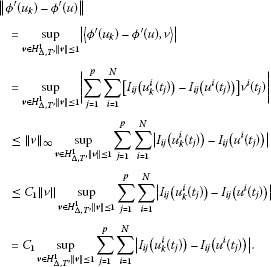The continuity of ${I}_{ij}$ and this imply that ${\varphi }^{\prime }\left({u}_{k}\right)\to {\varphi }^{\prime }\left(u\right)$ in ${H}_{\mathrm{\Delta },T}^{1}$. The proof is complete. □

First of all, we give two existence results.

Theorem 4.1 Suppose that (A) and the following conditions are satisfied.

(F1) ${lim}_{|x|\to \mathrm{\infty }}\frac{F\left(t,x\right)}{{|x|}^{2}}=+\mathrm{\infty }$ uniformly for Δ-a.e. $t\in {\left[0,T\right]}_{\mathbb{T}}$,

(F2) ${lim}_{|x|\to 0}\frac{F\left(t,x\right)}{{|x|}^{2}}=0$ uniformly for Δ-a.e. $t\in {\left[0,T\right]}_{\mathbb{T}}$,

(F3) there exist $\lambda >2$ and $\beta >\lambda -2$ such that

$\underset{|x|\to \mathrm{\infty }}{lim sup}\frac{F\left(t,x\right)}{{|x|}^{\lambda }}<\mathrm{\infty }\phantom{\rule{1em}{0ex}}\mathit{\text{uniformly for}}\phantom{\rule{0.5em}{0ex}}\mathrm{\Delta }\mathit{\text{-a.e.}}\phantom{\rule{0.5em}{0ex}}t\in {\left[0,T\right]}_{\mathbb{T}}$

and

$\underset{|x|\to \mathrm{\infty }}{lim inf}\frac{\left(\mathrm{\nabla }F\left(t,x\right),x\right)-2F\left(t,x\right)}{{|x|}^{\beta }}>0\phantom{\rule{1em}{0ex}}\mathit{\text{uniformly for}}\phantom{\rule{0.5em}{0ex}}\mathrm{\Delta }\mathit{\text{-a.e.}}\phantom{\rule{0.5em}{0ex}}t\in {\left[0,T\right]}_{\mathbb{T}},$

(F4) there exists $r>0$ such that

$F\left(t,x\right)\ge 0,\phantom{\rule{1em}{0ex}}\mathrm{\forall }|x|\le r,\phantom{\rule{0.5em}{0ex}}\mathit{\text{and}}\phantom{\rule{0.5em}{0ex}}\mathrm{\Delta }\phantom{\rule{0.5em}{0ex}}\mathit{\text{-a.e.}}\phantom{\rule{0.5em}{0ex}}t\in {\left[0,T\right]}_{\mathbb{T}},$

(F5) there exist ${a}_{ij},{b}_{ij}>0$ and ${\xi }_{ij}\in \left[0,1\right)$ such that

$|{I}_{ij}\left(t\right)|\le {a}_{ij}+{b}_{ij}{|t|}^{{\xi }_{ij}}\phantom{\rule{1em}{0ex}}\mathit{\text{for every}}\phantom{\rule{0.5em}{0ex}}t\in \mathbb{R},i\in \mathrm{\Gamma },j\in \mathrm{\Lambda },$

(F6) ${\int }_{0}^{t}{I}_{ij}\left(s\right)\phantom{\rule{0.2em}{0ex}}\mathrm{d}s\le 0$ for every $t\in \mathbb{R}$, $i\in \mathrm{\Gamma }$, $j\in \mathrm{\Lambda }$,

(F7) there exists ${\zeta }_{ij}>0$ such that

$2{\int }_{0}^{t}{I}_{ij}\left(s\right)\phantom{\rule{0.2em}{0ex}}\mathrm{d}s-{I}_{ij}\left(t\right)t\ge 0\phantom{\rule{1em}{0ex}}\mathit{\text{for all}}\phantom{\rule{0.5em}{0ex}}i\in \mathrm{\Gamma },j\in \mathrm{\Lambda }\phantom{\rule{0.5em}{0ex}}\mathit{\text{and}}\phantom{\rule{0.5em}{0ex}}|t|\ge {\zeta }_{ij},$

and

$\underset{t\to 0}{lim}\frac{{I}_{ij}\left(t\right)}{t}=0\phantom{\rule{1em}{0ex}}\mathit{\text{for all}}\phantom{\rule{0.5em}{0ex}}i\in \mathrm{\Gamma },j\in \mathrm{\Lambda }.$

Then problem (1.1) has at least two weak solutions. The one is a nontrivial weak solution, the other is a trivial weak solution.

Proof By Lemma 3.1, $\phi \in {C}^{1}\left(X,\mathbb{R}\right)$. Set $X={H}_{\mathrm{\Delta },T}^{1},{X}^{1}={H}^{+}$ with ${\left({e}_{n}\right)}_{n\ge 1}$ being its Hilbertian basis, ${X}^{2}={H}^{-}\oplus {H}^{0}$ and define

${X}_{n}^{1}=span\left\{{e}_{1},{e}_{2},\dots ,{e}_{n}\right\},\phantom{\rule{1em}{0ex}}n\in \mathbb{N},\phantom{\rule{2em}{0ex}}{X}_{n}^{2}={X}^{2},\phantom{\rule{1em}{0ex}}n\in \mathbb{N}.$

Then we have

${X}_{0}^{1}\subset {X}_{1}^{1}\subset \cdots \subset {X}^{1},\phantom{\rule{2em}{0ex}}{X}_{0}^{2}\subset {X}_{1}^{2}\subset \cdots \subset {X}^{2},\phantom{\rule{2em}{0ex}}{X}^{1}=\overline{\bigcup _{n\in \mathbb{N}}{X}_{n}^{1}},\phantom{\rule{2em}{0ex}}{X}^{2}=\overline{\bigcup _{n\in \mathbb{N}}{X}_{n}^{2}}$

and

$dim{X}_{n}^{1}<+\mathrm{\infty },\phantom{\rule{2em}{0ex}}dim{X}_{n}^{2}<+\mathrm{\infty },\phantom{\rule{1em}{0ex}}n\in \mathbb{N}.$

We divide our proof into four parts in order to show Theorem 4.1.

Firstly, we show that φ satisfies the ${\left(C\right)}^{\ast }$ condition.

Let $\left\{{u}_{{\alpha }_{n}}\right\}$ be a sequence in ${H}_{\mathrm{\Delta },T}^{1}$ such that ${\alpha }_{n}$ is admissible and

${u}_{{\alpha }_{n}}\in {X}_{{\alpha }_{n}},sup|\phi \left({u}_{{\alpha }_{n}}\right)|<+\mathrm{\infty },\phantom{\rule{1em}{0ex}}\left(1+\parallel {u}_{{\alpha }_{n}}\parallel \right){\phi }^{\prime }\left({u}_{{\alpha }_{n}}\right)\to 0,$

then there exists a constant ${C}_{2}>0$ such that

$|\phi \left({u}_{{\alpha }_{n}}\right)|\le {C}_{2},\phantom{\rule{1em}{0ex}}\left(1+\parallel {u}_{{\alpha }_{n}}\parallel \right){\phi }^{\prime }\left({u}_{{\alpha }_{n}}\right)\le {C}_{2}$
(4.1)

for all large n. On the other hand, by (F3), there are constants ${C}_{3}>0$ and ${\rho }_{1}>0$ such that

$F\left(t,x\right)\le {C}_{3}{|x|}^{\lambda }$
(4.2)

for all $|x|\ge {\rho }_{1}$ and Δ-a.e. $t\in {\left[0,T\right]}_{\mathbb{T}}$. By (A) one has

$|F\left(t,x\right)|\le \underset{s\in \left[0,{\rho }_{1}\right]}{max}a\left(s\right)b\left(t\right)$
(4.3)

for all $|x|\le {\rho }_{1}$ and Δ-a.e. $t\in {\left[0,T\right]}_{\mathbb{T}}$. It follows from (4.2) and (4.3) that

$|F\left(t,x\right)|\le \underset{s\in \left[0,{\rho }_{1}\right]}{max}a\left(s\right)b\left(t\right)+{C}_{3}{|x|}^{\lambda }$
(4.4)

for all $x\in {\mathbb{R}}^{N}$ and Δ-a.e. $t\in {\left[0,T\right]}_{\mathbb{T}}$. Since ${d}_{lm}\in {L}^{\mathrm{\infty }}\left(\left[0,T\right]\right)$ for all $l,m=1,2,\dots ,N$, there exists a constant ${C}_{4}\ge 1$ such that

$|{\int }_{{\left[0,T\right)}_{\mathbb{T}}}\left({A}^{\sigma }\left(t\right){u}^{\sigma }\left(t\right),{u}^{\sigma }\left(t\right)\right)\mathrm{\Delta }t|\le {C}_{4}{\int }_{{\left[0,T\right)}_{\mathbb{T}}}{|{u}^{\sigma }\left(t\right)|}^{2}\mathrm{\Delta }t,\phantom{\rule{1em}{0ex}}\mathrm{\forall }u\in {H}_{\mathrm{\Delta },T}^{1}.$
(4.5)

From (F5) and (2.5), we have that

$\begin{array}{rcl}|\varphi \left(u\right)|& \le & \sum _{j=1}^{p}\sum _{i=1}^{N}{\int }_{0}^{|{u}^{i}\left({t}_{j}\right)|}\left({a}_{ij}+{b}_{ij}{|t|}^{{\xi }_{ij}}\right)\phantom{\rule{0.2em}{0ex}}\mathrm{d}t\\ \le & \overline{a}pN{\parallel u\parallel }_{\mathrm{\infty }}+\overline{b}\sum _{j=1}^{p}\sum _{i=1}^{N}{\parallel u\parallel }_{\mathrm{\infty }}^{{\xi }_{ij}+1}\\ \le & \overline{a}pN{C}_{1}\parallel u\parallel +\overline{b}{C}_{1}\sum _{j=1}^{p}\sum _{i=1}^{N}{\parallel u\parallel }^{{\xi }_{ij}+1}\end{array}$
(4.6)

for all $u\in {H}_{\mathrm{\Delta },T}^{1}$, where $\overline{a}={max}_{i\in \mathrm{\Gamma },j\in \mathrm{\Lambda }}\left\{{a}_{ij}\right\}$, $\overline{b}={max}_{i\in \mathrm{\Gamma },j\in \mathrm{\Lambda }}\left\{{b}_{ij}\right\}$. Combining (4.4), (4.5), (4.6) and Hölder’s inequality, we have

$\begin{array}{rcl}\frac{1}{2}{\parallel {u}_{{\alpha }_{n}}\parallel }^{2}& =& \phi \left({u}_{{\alpha }_{n}}\right)-\varphi \left({u}_{{\alpha }_{n}}\right)+\frac{1}{2}{\int }_{{\left[0,T\right)}_{\mathbb{T}}}{|{u}_{{\alpha }_{n}}^{\sigma }\left(t\right)|}^{2}\mathrm{\Delta }t\\ +\frac{1}{2}{\int }_{{\left[0,T\right)}_{\mathbb{T}}}\left({A}^{\sigma }\left(t\right){u}_{{\alpha }_{n}}^{\sigma }\left(t\right),{u}_{{\alpha }_{n}}^{\sigma }\left(t\right)\right)\mathrm{\Delta }t-J\left({u}_{{\alpha }_{n}}\right)\\ \le & {C}_{2}+\overline{a}pN{C}_{1}\parallel {u}_{{\alpha }_{n}}\parallel +\overline{b}{C}_{1}\sum _{j=1}^{p}\sum _{i=1}^{N}{\parallel {u}_{{\alpha }_{n}}\parallel }^{{\xi }_{ij}+1}+{C}_{4}{\int }_{{\left[0,T\right)}_{\mathbb{T}}}{|{u}_{{\alpha }_{n}}^{\sigma }\left(t\right)|}^{2}\mathrm{\Delta }t\\ +{C}_{3}{\int }_{{\left[0,T\right)}_{\mathbb{T}}}{|{u}_{{\alpha }_{n}}^{\sigma }\left(t\right)|}^{\lambda }\mathrm{\Delta }t+\underset{s\in \left[0,{\rho }_{1}\right]}{max}a\left(s\right){\int }_{{\left[0,T\right)}_{\mathbb{T}}}{b}^{\sigma }\left(t\right)\mathrm{\Delta }t\\ \le & {C}_{2}+\overline{a}pN{C}_{1}\parallel {u}_{{\alpha }_{n}}\parallel +\overline{b}{C}_{1}\sum _{j=1}^{p}\sum _{i=1}^{N}{\parallel {u}_{{\alpha }_{n}}\parallel }^{{\xi }_{ij}+1}\\ +{C}_{4}{T}^{\frac{\lambda -2}{\lambda }}{\left({\int }_{{\left[0,T\right)}_{\mathbb{T}}}{|{u}_{{\alpha }_{n}}^{\sigma }\left(t\right)|}^{\lambda }\mathrm{\Delta }t\right)}^{\frac{2}{\lambda }}+{C}_{3}{\int }_{{\left[0,T\right)}_{\mathbb{T}}}{|{u}_{{\alpha }_{n}}^{\sigma }\left(t\right)|}^{\lambda }\mathrm{\Delta }t+{C}_{5}\end{array}$
(4.7)

for all large n, where ${C}_{5}={max}_{s\in \left[0,{\rho }_{1}\right]}a\left(s\right){\int }_{{\left[0,T\right)}_{\mathbb{T}}}{b}^{\sigma }\left(t\right)\mathrm{\Delta }t$. On the other hand, by (F3), there exist ${C}_{6}>0$ and ${\rho }_{2}>0$ such that

$\left(\mathrm{\nabla }F\left(t,x\right),x\right)-2F\left(t,x\right)\ge {C}_{6}{|x|}^{\beta }$
(4.8)

for all $|x|\ge {\rho }_{2}$ and Δ-a.e. $t\in {\left[0,T\right]}_{\mathbb{T}}$. By (A),

$|\left(\mathrm{\nabla }F\left(t,x\right),x\right)-2F\left(t,x\right)|\le {C}_{7}b\left(t\right)$
(4.9)

for all $|x|\le {\rho }_{2}$ and Δ-a.e. $t\in {\left[0,T\right]}_{\mathbb{T}}$, where ${C}_{7}=\left(2+{\rho }_{2}\right){max}_{s\in \left[0,{\rho }_{2}\right]}a\left(s\right)$. Combining (4.8) and (4.9), one has

$\left(\mathrm{\nabla }F\left(t,x\right),x\right)-2F\left(t,x\right)\ge {C}_{6}{|x|}^{\beta }-{C}_{6}{\rho }_{2}^{\beta }-{C}_{7}b\left(t\right)$
(4.10)

for all $x\in {\mathbb{R}}^{N}$ and Δ-a.e. $t\in {\left[0,T\right]}_{\mathbb{T}}$. According to (F7), there exists ${C}_{8}>0$ such that

(4.11)

Thus, by (4.1), (4.10) and (4.11), we obtain

$\begin{array}{rcl}3{C}_{2}& \ge & 2\phi \left({u}_{{\alpha }_{n}}\right)-〈{\phi }^{\prime }\left({u}_{{\alpha }_{n}}\right),{u}_{{\alpha }_{n}}〉\\ =& 2\varphi \left({u}_{{\alpha }_{n}}\right)-〈{\varphi }^{\prime }\left({u}_{{\alpha }_{n}}\right),{u}_{{\alpha }_{n}}〉\\ +{\int }_{{\left[0,T\right)}_{\mathbb{T}}}\left[\left(\mathrm{\nabla }F\left(\sigma \left(t\right),{u}_{{\alpha }_{n}}^{\sigma }\left(t\right)\right),{u}_{{\alpha }_{n}}^{\sigma }\left(t\right)\right)-2F\left(\sigma \left(t\right),{u}_{{\alpha }_{n}}^{\sigma }\left(t\right)\right)\right]\mathrm{\Delta }t\\ =& \sum _{j=1}^{p}\sum _{i=1}^{N}\left(2{\int }_{0}^{{u}_{{\alpha }_{n}}^{i}\left({t}_{j}\right)}{I}_{ij}\left(t\right)\phantom{\rule{0.2em}{0ex}}\mathrm{d}t-{I}_{ij}\left({u}_{{\alpha }_{n}}^{i}\left({t}_{j}\right)\right){u}_{{\alpha }_{n}}^{i}\left({t}_{j}\right)\right)\\ +{\int }_{{\left[0,T\right)}_{\mathbb{T}}}\left[\left(\mathrm{\nabla }F\left(\sigma \left(t\right),{u}_{{\alpha }_{n}}^{\sigma }\left(t\right)\right),{u}_{{\alpha }_{n}}^{\sigma }\left(t\right)\right)-2F\left(\sigma \left(t\right),{u}_{{\alpha }_{n}}^{\sigma }\left(t\right)\right)\right]\mathrm{\Delta }t\\ \ge & -pN{C}_{8}+{C}_{6}{\int }_{{\left[0,T\right)}_{\mathbb{T}}}{|{u}_{{\alpha }_{n}}^{\sigma }|}^{\beta }\mathrm{\Delta }t-{C}_{6}{\rho }_{2}^{\beta }T-{C}_{7}{\int }_{{\left[0,T\right)}_{\mathbb{T}}}{b}^{\sigma }\left(t\right)\mathrm{\Delta }t\end{array}$
(4.12)

for all large n. From (4.12), ${\int }_{{\left[0,T\right)}_{\mathbb{T}}}{|{u}_{{\alpha }_{n}}^{\sigma }|}^{\beta }\mathrm{\Delta }t$ is bounded. If $\beta >\lambda$, by Hölder’s inequality, we have

${\int }_{{\left[0,T\right)}_{\mathbb{T}}}{|{u}_{{\alpha }_{n}}^{\sigma }\left(t\right)|}^{\lambda }\mathrm{\Delta }t\le {T}^{\frac{\beta -\lambda }{\beta }}{\left({\int }_{{\left[0,T\right)}_{\mathbb{T}}}{|{u}_{{\alpha }_{n}}^{\sigma }\left(t\right)|}^{\beta }\mathrm{\Delta }t\right)}^{\frac{\lambda }{\beta }}.$
(4.13)

Since ${\xi }_{ij}\in \left[0,1\right)$ for all $i\in \mathrm{\Gamma }$, $j\in \mathrm{\Lambda }$, by (4.7) and (4.13), $\left\{{u}_{{\alpha }_{n}}\right\}$ is bounded in ${H}_{\mathrm{\Delta },T}^{1}$. If $\beta \le \lambda$, by (2.5), we obtain

$\begin{array}{rcl}{\int }_{{\left[0,T\right)}_{\mathbb{T}}}{|{u}_{{\alpha }_{n}}^{\sigma }\left(t\right)|}^{\lambda }\mathrm{\Delta }t& =& {\int }_{{\left[0,T\right)}_{\mathbb{T}}}{|{u}_{{\alpha }_{n}}^{\sigma }\left(t\right)|}^{\beta }{|{u}_{{\alpha }_{n}}^{\sigma }\left(t\right)|}^{\lambda -\beta }\mathrm{\Delta }t\\ \le & {\parallel {u}_{{\alpha }_{n}}\parallel }_{\mathrm{\infty }}^{\lambda -\beta }{\int }_{{\left[0,T\right)}_{\mathbb{T}}}{|{u}_{{\alpha }_{n}}^{\sigma }\left(t\right)|}^{\beta }\mathrm{\Delta }t\\ \le & {C}_{1}^{\lambda -\beta }{\parallel {u}_{{\alpha }_{n}}\parallel }^{\lambda -\beta }{\int }_{{\left[0,T\right)}_{\mathbb{T}}}{|{u}_{{\alpha }_{n}}^{\sigma }\left(t\right)|}^{\beta }\mathrm{\Delta }t.\end{array}$
(4.14)

Since ${\xi }_{ij}\in \left[0,1\right)$, $\lambda -\beta <2$, by (4.7) and (4.14), $\left\{{u}_{{\alpha }_{n}}\right\}$ is also bounded in ${H}_{\mathrm{\Delta },T}^{1}$. Hence, $\left\{{u}_{{\alpha }_{n}}\right\}$ is also bounded in ${H}_{\mathrm{\Delta },T}^{1}$. Going if necessary to a subsequence, we can assume that ${u}_{{\alpha }_{n}}⇀u$ in ${H}_{\mathrm{\Delta },T}^{1}$. From Theorem 2.5, we have ${\parallel {u}_{{\alpha }_{n}}-u\parallel }_{\mathrm{\infty }}\to 0$ and ${\int }_{{\left[0,T\right)}_{\mathbb{T}}}{|{u}_{{\alpha }_{n}}^{\sigma }\left(t\right)-{u}^{\sigma }\left(t\right)|}^{2}\mathrm{\Delta }t\to 0$. Since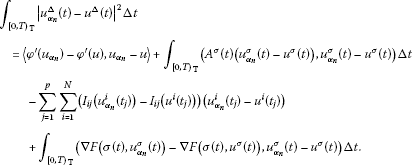This implies ${\int }_{{\left[0,T\right)}_{\mathbb{T}}}{|{u}_{{\alpha }_{n}}^{\mathrm{\Delta }}\left(t\right)-{u}^{\mathrm{\Delta }}\left(t\right)|}^{2}\mathrm{\Delta }t\to 0$, and hence $\parallel {u}_{{\alpha }_{n}}-u\parallel \to 0$. Therefore, ${u}_{{\alpha }_{n}}\to u$ in ${H}_{\mathrm{\Delta },T}^{1}$. Hence φ satisfies the ${\left(C\right)}^{\ast }$ condition.

Secondly, we show that φ maps bounded sets into bounded sets.

It follows from (3.2), (4.4), (4.5) and (4.6) that

$\begin{array}{rcl}|\phi \left(u\right)|& =& \frac{1}{2}{\int }_{{\left[0,T\right)}_{\mathbb{T}}}{|{u}^{\mathrm{\Delta }}\left(t\right)|}^{2}\mathrm{\Delta }t+\sum _{j=1}^{p}\sum _{i=1}^{N}{\int }_{0}^{{u}^{i}\left({t}_{j}\right)}{I}_{ij}\left(t\right)\phantom{\rule{0.2em}{0ex}}\mathrm{d}t\\ -\frac{1}{2}{\int }_{{\left[0,T\right)}_{\mathbb{T}}}\left({A}^{\sigma }\left(t\right){u}^{\sigma }\left(t\right),{u}^{\sigma }\left(t\right)\right)\mathrm{\Delta }t+J\left(u\right)\\ \le & \frac{1}{2}{\int }_{{\left[0,T\right)}_{\mathbb{T}}}{|{u}^{\mathrm{\Delta }}\left(t\right)|}^{2}\mathrm{\Delta }t+\frac{{C}_{4}}{2}{\int }_{{\left[0,T\right)}_{\mathbb{T}}}{|{u}^{\sigma }\left(t\right)|}^{2}\mathrm{\Delta }t+\overline{a}pN{C}_{1}\parallel u\parallel \\ +\overline{b}{C}_{1}\sum _{j=1}^{p}\sum _{i=1}^{N}{\parallel u\parallel }^{{\xi }_{ij}+1}\\ +{C}_{3}{\int }_{{\left[0,T\right)}_{\mathbb{T}}}{|{u}^{\sigma }\left(t\right)|}^{\lambda }\mathrm{\Delta }t+\underset{s\in \left[0,{\rho }_{1}\right]}{max}a\left(s\right){\int }_{{\left[0,T\right)}_{\mathbb{T}}}{b}^{\sigma }\left(t\right)\mathrm{\Delta }t\\ \le & \frac{1}{2}{C}_{4}{\parallel u\parallel }^{2}+\overline{a}pN{C}_{1}\parallel u\parallel +\overline{b}{C}_{1}\sum _{j=1}^{p}\sum _{i=1}^{N}{\parallel u\parallel }^{{\xi }_{ij}+1}+{C}_{3}T{\parallel u\parallel }_{\mathrm{\infty }}^{\lambda }+{C}_{5}\\ \le & \frac{1}{2}{C}_{4}{\parallel u\parallel }^{2}+\overline{a}pN{C}_{1}\parallel u\parallel +\overline{b}{C}_{1}\sum _{j=1}^{p}\sum _{i=1}^{N}{\parallel u\parallel }^{{\xi }_{ij}+1}+{C}_{3}T{C}_{1}^{\lambda }{\parallel u\parallel }^{\lambda }+{C}_{5}\end{array}$

for all $u\in {H}_{\mathrm{\Delta },T}^{1}$. Thus, φ maps bounded sets into bounded sets.

Thirdly, we claim that φ has a local linking at 0 with respect to $\left({X}^{1},{X}^{2}\right)$.

Applying (F2), for ${ϵ}_{1}=\frac{\delta }{4}$, there exists ${\rho }_{3}>0$ such that

$|F\left(t,x\right)|\le {ϵ}_{1}{|x|}^{2}$
(4.15)

for all $|x|\le {\rho }_{3}$ and Δ-a.e. $t\in {\left[0,T\right]}_{\mathbb{T}}$. By (F7), for ${ϵ}_{2}=\frac{\delta }{4pN{C}_{1}}$, there exists ${\rho }_{4}>0$ such that

$|{I}_{ij}\left(t\right)|\le {ϵ}_{2}|t|,\phantom{\rule{1em}{0ex}}|t|\le {\rho }_{4},i\in \mathrm{\Gamma },j\in \mathrm{\Lambda }.$
(4.16)

Let ${\rho }_{5}=min\left\{{\rho }_{3},{\rho }_{4}\right\}$. For $u\in {X}^{1}$ with $\parallel u\parallel \le {r}_{1}\triangleq \frac{{\rho }_{5}}{{C}_{1}}$, by (2.5), (3.2), (3.6), (4.15) and (4.16), we have

$\begin{array}{rcl}\phi \left(u\right)& =& q\left(u\right)+\sum _{j=1}^{p}\sum _{i=1}^{N}{\int }_{0}^{{u}^{i}\left({t}_{j}\right)}{I}_{ij}\left(t\right)\phantom{\rule{0.2em}{0ex}}\mathrm{d}t-{\int }_{{\left[0,T\right)}_{\mathbb{T}}}F\left(\sigma \left(t\right),{u}^{\sigma }\left(t\right)\right)\mathrm{\Delta }t\\ \ge & \delta {\parallel u\parallel }^{2}-\sum _{j=1}^{p}\sum _{i=1}^{N}{\int }_{0}^{|{u}^{i}\left({t}_{j}\right)|}|{I}_{ij}\left(t\right)|\phantom{\rule{0.2em}{0ex}}\mathrm{d}t-{ϵ}_{1}{\int }_{{\left[0,T\right)}_{\mathbb{T}}}{|{u}^{\sigma }\left(t\right)|}^{2}\mathrm{\Delta }t\\ \ge & \delta {\parallel u\parallel }^{2}-\sum _{j=1}^{p}\sum _{i=1}^{N}{\int }_{0}^{|{u}^{i}\left({t}_{j}\right)|}{ϵ}_{2}|t|\phantom{\rule{0.2em}{0ex}}\mathrm{d}t-{ϵ}_{1}{\int }_{{\left[0,T\right)}_{\mathbb{T}}}{|{u}^{\sigma }\left(t\right)|}^{2}\mathrm{\Delta }t\\ \ge & \delta {\parallel u\parallel }^{2}-{ϵ}_{2}\sum _{j=1}^{p}\sum _{i=1}^{N}{\parallel u\parallel }_{\mathrm{\infty }}^{2}-{ϵ}_{1}{\int }_{{\left[0,T\right)}_{\mathbb{T}}}{|{u}^{\sigma }\left(t\right)|}^{2}\mathrm{\Delta }t\\ \ge & \delta {\parallel u\parallel }^{2}-{ϵ}_{2}pN{C}_{1}{\parallel u\parallel }^{2}-{ϵ}_{1}{\parallel u\parallel }^{2}\\ \ge & \delta {\parallel u\parallel }^{2}-\frac{\delta }{4}{\parallel u\parallel }^{2}-\frac{\delta }{4}{\parallel u\parallel }^{2}\\ =& \frac{\delta }{2}{\parallel u\parallel }^{2}.\end{array}$

This implies that

On the other hand, it follows from (F6) that

$\varphi \left(u\right)\le 0$
(4.17)

for all $u\in {H}_{\mathrm{\Delta },T}^{1}$. Let $u={u}^{-}+{u}^{0}\in {X}^{2}$ satisfy $\parallel u\parallel \le {r}_{2}\triangleq \frac{r}{{C}_{1}}$. Using (F4), (2.5), (3.2), (3.5) and (4.17), we obtain

$\begin{array}{rcl}\phi \left(u\right)& =& q\left(u\right)+\varphi \left(u\right)-{\int }_{{\left[0,T\right)}_{\mathbb{T}}}F\left(\sigma \left(t\right),{u}^{\sigma }\left(t\right)\right)\mathrm{\Delta }t\\ \le & -\delta {\parallel {u}^{-}\parallel }^{2}-{\int }_{{\left[0,T\right)}_{\mathbb{T}}}F\left(\sigma \left(t\right),{u}^{\sigma }\left(t\right)\right)\mathrm{\Delta }t\\ \le & -\delta {\parallel {u}^{-}\parallel }^{2}.\end{array}$

This implies that

Let $M=min\left\{{r}_{1},{r}_{2}\right\}$. Then φ satisfies the condition $\left({\mathrm{I}}_{1}\right)$ of Theorem 3.1.

Finally, we claim that for every $n\in \mathbb{N}$,

For given $n\in \mathbb{N}$, since ${X}_{n}^{1}\oplus {X}^{2}$ is a finite dimensional space, there exists ${C}_{9}>0$ such that

$\parallel u\parallel \le {C}_{9}{\parallel u\parallel }_{{L}^{2}},\phantom{\rule{1em}{0ex}}\mathrm{\forall }u\in {X}_{n}^{1}\oplus {X}^{2}.$
(4.18)

By (F1), there exists ${\rho }_{6}>0$ such that

$F\left(t,x\right)\ge {C}_{9}^{2}\left({C}_{4}+\delta \right){|x|}^{2}$
(4.19)

for all $|x|\ge {\rho }_{6}$ and Δ-a.e. $t\in {\left[0,T\right]}_{\mathbb{T}}$. From (A), we get

$|F\left(t,x\right)|\le \underset{s\in \left[0,{\rho }_{6}\right]}{max}a\left(s\right)b\left(t\right)$
(4.20)

for all $|x|\le {\rho }_{6}$ and Δ-a.e. $t\in {\left[0,T\right]}_{\mathbb{T}}$. Equations (4.19) and (4.20) imply that

$F\left(t,x\right)\ge {C}_{9}^{2}\left({C}_{4}+\delta \right){|x|}^{2}-{C}_{10}-\underset{s\in \left[0,{\rho }_{6}\right]}{max}a\left(s\right)b\left(t\right)$
(4.21)

for all $x\in {\mathbb{R}}^{N}$ and Δ-a.e. $t\in {\left[0,T\right]}_{\mathbb{T}}$, where ${C}_{10}={C}_{9}^{2}\left({C}_{4}+\delta \right){\rho }_{6}^{2}$. Using (3.2), (3.6), (4.5), (4.17), (4.18) and (4.21), we have, for $u={u}^{+}+{u}^{0}+{u}^{-}\in {X}_{n}^{1}\oplus {X}^{2}={X}_{n}^{1}\oplus {H}^{0}\oplus {H}^{-}$,

$\begin{array}{rcl}\phi \left(u\right)& =& \frac{1}{2}{\int }_{{\left[0,T\right)}_{\mathbb{T}}}{|{u}^{\mathrm{\Delta }}\left(t\right)|}^{2}\mathrm{\Delta }t+\sum _{j=1}^{p}\sum _{i=1}^{N}{\int }_{0}^{{u}^{i}\left({t}_{j}\right)}{I}_{ij}\left(t\right)\phantom{\rule{0.2em}{0ex}}\mathrm{d}t\\ -\frac{1}{2}{\int }_{{\left[0,T\right)}_{\mathbb{T}}}\left({A}^{\sigma }\left(t\right){u}^{\sigma }\left(t\right),{u}^{\sigma }\left(t\right)\right)\mathrm{\Delta }t-{\int }_{{\left[0,T\right)}_{\mathbb{T}}}F\left(\sigma \left(t\right),{u}^{\sigma }\left(t\right)\right)\mathrm{\Delta }t\\ \le & -\delta {\parallel {u}^{-}\parallel }^{2}+\frac{1}{2}{\int }_{{\left[0,T\right)}_{\mathbb{T}}}{|{\left({u}^{+}\right)}^{\mathrm{\Delta }}\left(t\right)|}^{2}\mathrm{\Delta }t\\ -\frac{1}{2}{\int }_{{\left[0,T\right)}_{\mathbb{T}}}\left({A}^{\sigma }\left(t\right){\left({u}^{+}\right)}^{\sigma }\left(t\right),{\left({u}^{+}\right)}^{\sigma }\left(t\right)\right)\mathrm{\Delta }t-{\int }_{{\left[0,T\right)}_{\mathbb{T}}}F\left(\sigma \left(t\right),{u}^{\sigma }\left(t\right)\right)\mathrm{\Delta }t\\ \le & -\delta {\parallel {u}^{-}\parallel }^{2}+\frac{1}{2}{\int }_{{\left[0,T\right)}_{\mathbb{T}}}{|{\left({u}^{+}\right)}^{\mathrm{\Delta }}\left(t\right)|}^{2}\mathrm{\Delta }t+\frac{{C}_{4}}{2}{\int }_{{\left[0,T\right)}_{\mathbb{T}}}{|{\left({u}^{+}\right)}^{\sigma }\left(t\right)|}^{2}\mathrm{\Delta }t\\ -{\int }_{{\left[0,T\right)}_{\mathbb{T}}}F\left(\sigma \left(t\right),{u}^{\sigma }\left(t\right)\right)\mathrm{\Delta }t\\ \le & -\delta {\parallel {u}^{-}\parallel }^{2}+\frac{{C}_{4}}{2}{\parallel {u}^{+}\parallel }^{2}-{C}_{9}^{2}\left({C}_{4}+\delta \right){\parallel {u}^{\sigma }\parallel }_{{L}^{2}}^{2}+{C}_{10}T+\underset{s\in \left[0,{\rho }_{6}\right]}{max}a\left(s\right){\int }_{{\left[0,T\right)}_{\mathbb{T}}}{b}^{\sigma }\left(t\right)\mathrm{\Delta }t\\ \le & -\delta {\parallel {u}^{-}\parallel }^{2}+{C}_{4}{\parallel {u}^{+}\parallel }^{2}-\left({C}_{4}+\delta \right){\parallel u\parallel }^{2}+{C}_{10}T+{C}_{11}\\ =& -\delta {\parallel {u}^{-}\parallel }^{2}+{C}_{4}{\parallel {u}^{+}\parallel }^{2}-\left({C}_{4}+\delta \right){\parallel {u}^{+}+{u}^{0}+{u}^{-}\parallel }^{2}+{C}_{10}T+{C}_{11}\\ \le & -\delta {\parallel {u}^{-}\parallel }^{2}+{C}_{4}{\parallel {u}^{+}\parallel }^{2}-\left({C}_{4}+\delta \right){\parallel {u}^{+}\parallel }^{2}-\delta {\parallel {u}^{0}+{u}^{-}\parallel }^{2}+{C}_{10}T+{C}_{11}\\ \le & -\delta {\parallel {u}^{-}\parallel }^{2}+{C}_{4}{\parallel {u}^{+}\parallel }^{2}-\left({C}_{4}+\delta \right){\parallel {u}^{+}\parallel }^{2}-\delta {\parallel {u}^{0}\parallel }^{2}+{C}_{10}T+{C}_{11}\\ =& -\delta {\parallel u\parallel }^{2}+{C}_{10}T+{C}_{11},\end{array}$

where ${C}_{11}={max}_{s\in \left[0,{\rho }_{6}\right]}a\left(s\right){\int }_{{\left[0,T\right)}_{\mathbb{T}}}{b}^{\sigma }\left(t\right)\mathrm{\Delta }t$. Hence, for every $n\in \mathbb{N}$, $\phi \left(u\right)\to -\mathrm{\infty }$ as $\parallel u\parallel \to \mathrm{\infty }$ and ${X}_{n}^{1}\oplus {X}^{2}$.

Thus, by Theorem 3.1, problem (1.1) has at least one nontrivial weak solution. The proof is complete. □

Example 4.1 Let $\mathbb{T}=\mathbb{R}$, $T=\frac{\pi }{2}$, $N=1$, ${t}_{1}=\frac{\pi }{4}$. Consider the second-order Hamiltonian system with impulsive effects

(4.22)

where $A\left(t\right)=1$,

$F\left(t,x\right)=\left\{\begin{array}{cc}{|x|}^{4},\hfill & |x|\ge 5,\hfill \\ \frac{625}{5-3\sqrt{2}}x-\frac{1875\sqrt{2}}{5-3\sqrt{2}},\hfill & 3\sqrt{2}

for all $x\in \mathbb{R}$ and $t\in \left[0,\frac{\pi }{2}\right]$,

$I\left(t\right)=\left\{\begin{array}{cc}0,\hfill & t\ge 4,\hfill \\ -6{\left(t-4\right)}^{3},\hfill & 3\le t<4,\hfill \\ 6t-12,\hfill & 1

then all conditions of Theorem 4.1 hold. According to Theorem 4.1, problem (4.22) has at least one nontrivial weak solution. In fact,

$u\left(t\right)=\left\{\begin{array}{cc}3\sqrt{2}cost,\hfill & t\in \left[0,\frac{\pi }{4}\right];\hfill \\ 3\sqrt{2}sint,\hfill & t\in \left[\frac{\pi }{4},\frac{\pi }{2}\right]\hfill \end{array}$

is the solution of problem (4.22).

Theorem 4.2 Assume that (A), (F5), (F6), (F7) and the following conditions are satisfied.

(F8) ${lim sup}_{|x|\to 0}\frac{F\left(t,x\right)}{{|x|}^{2}}\le 0$ uniformly for Δ-a.e. $t\in {\left[0,T\right]}_{\mathbb{T}}$,

(F9) there exist constants $\mu >2$ and ${r}_{3}\ge 0$ such that $\left(\mathrm{\nabla }F\left(t,x\right),x\right)\ge \mu F\left(t,x\right)>0$ for all $t\in {\left[0,T\right]}_{\mathbb{T}}$ and $|x|\ge {r}_{3}$,

(F10) $F\left(t,x\right)\ge 0$ for all $x\in {\mathbb{R}}^{N}$ and Δ-a.e. $t\in {\left[0,T\right]}_{\mathbb{T}}$.

Then problem (1.1) has at least one nontrivial weak solution.

Proof Set ${E}_{1}={H}^{+}$, ${E}_{2}={H}^{-}\oplus {H}^{0}$ and $E={H}_{\mathrm{\Delta },T}^{1}$. Then E is a real Hilbert space, $E={E}_{1}\oplus {E}_{2}$, ${E}_{2}={E}_{1}^{\mathrm{\perp }}$ and $dim\left({E}_{2}\right)<+\mathrm{\infty }$.

Firstly, we prove that φ satisfies the (PS) condition. Indeed, let $\left\{{u}_{k}\right\}\subset {H}_{\mathrm{\Delta },T}^{1}$ be a sequence such that $|\phi \left({u}_{k}\right)|\le {C}_{12}$ and ${\phi }^{\prime }\left({u}_{k}\right)\to 0$ as $k\to \mathrm{\infty }$. As the proof of Theorem 4.1, it suffices to show that $\left\{{u}_{k}\right\}$ is bounded in ${H}_{\mathrm{\Delta },T}^{1}$. By (F9) there exist positive constants ${C}_{13}$, ${C}_{14}$ such that

$F\left(t,x\right)\ge {C}_{13}{|x|}^{\mu }-{C}_{14},\phantom{\rule{1em}{0ex}}\mathrm{\forall }t\in {\left[0,T\right]}_{\mathbb{T}},\mathrm{\forall }x\in {\mathbb{R}}^{n}$
(4.23)

(see ). By (F9), (4.11) and (4.23), we have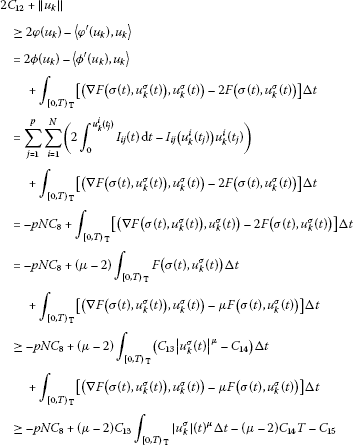(4.24)

for large k, where ${C}_{15}=\left({r}_{3}+\mu \right){max}_{s\in \left[0,{r}_{3}\right]}a\left(s\right){\int }_{{\left[0,T\right)}_{\mathbb{T}}}{b}^{\sigma }\left(t\right)\mathrm{\Delta }t$. Equation (4.24) implies that there exists ${C}_{16}>0$ such that

${\int }_{{\left[0,T\right)}_{\mathbb{T}}}{|{u}_{k}^{\sigma }\left(t\right)|}^{\mu }\mathrm{\Delta }t\le {C}_{16}\left(1+\parallel {u}_{k}\parallel \right).$
(4.25)

Combining (3.2), (4.6), (4.11) and (4.25), we obtain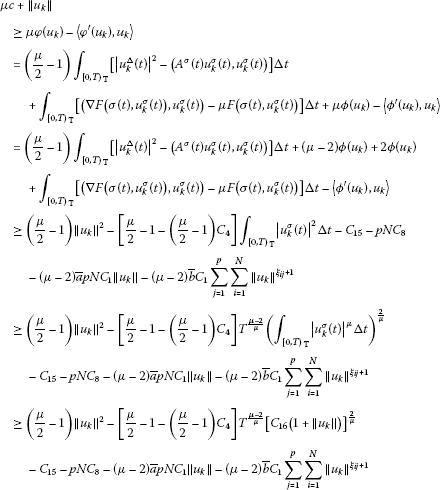(4.26)

for large k. Since $\mu >2$, ${\xi }_{ij}\in \left[0,1\right)$, by (4.26), $\left\{{u}_{k}\right\}$ is bounded in ${H}_{\mathrm{\Delta },T}^{1}$.

For any small ${ϵ}_{3}=\frac{\delta }{2}$, by (F8) we know that there is a ${\rho }_{7}>0$ such that

(4.27)

By (F7), for ${ϵ}_{4}=\frac{\delta }{8pN{C}_{1}}$, there exists ${\rho }_{8}>0$ such that

$|{I}_{ij}\left(t\right)|\le {ϵ}_{4}|t|,\phantom{\rule{1em}{0ex}}|t|\le {\rho }_{8},i\in \mathrm{\Gamma },j\in \mathrm{\Lambda }.$
(4.28)

Let ${\rho }_{9}=\frac{1}{2}min\left\{{\rho }_{7},{\rho }_{8}\right\}$. For $u\in {E}^{1}$ with $\parallel u\parallel \le {r}_{1}\triangleq \frac{{\rho }_{9}}{{C}_{1}}$, by (2.5), (3.2), (3.6), (4.27) and (4.28), we have

$\begin{array}{rcl}\phi \left(u\right)& =& q\left(u\right)+\sum _{j=1}^{p}\sum _{i=1}^{N}{\int }_{0}^{{u}^{i}\left({t}_{j}\right)}{I}_{ij}\left(t\right)\phantom{\rule{0.2em}{0ex}}\mathrm{d}t-{\int }_{{\left[0,T\right)}_{\mathbb{T}}}F\left(\sigma \left(t\right),{u}^{\sigma }\left(t\right)\right)\mathrm{\Delta }t\\ \ge & \delta {\parallel u\parallel }^{2}-\sum _{j=1}^{p}\sum _{i=1}^{N}{\int }_{0}^{|{u}^{i}\left({t}_{j}\right)|}|{I}_{ij}\left(t\right)|\phantom{\rule{0.2em}{0ex}}\mathrm{d}t-{ϵ}_{3}{\int }_{{\left[0,T\right)}_{\mathbb{T}}}{|{u}^{\sigma }\left(t\right)|}^{2}\mathrm{\Delta }t\\ \ge & \delta {\parallel u\parallel }^{2}-\sum _{j=1}^{p}\sum _{i=1}^{N}{\int }_{0}^{|{u}^{i}\left({t}_{j}\right)|}{ϵ}_{4}|t|\phantom{\rule{0.2em}{0ex}}\mathrm{d}t-{ϵ}_{3}{\int }_{{\left[0,T\right)}_{\mathbb{T}}}{|{u}^{\sigma }\left(t\right)|}^{2}\mathrm{\Delta }t\\ \ge & \delta {\parallel u\parallel }^{2}-{ϵ}_{4}\sum _{j=1}^{p}\sum _{i=1}^{N}{\parallel u\parallel }_{\mathrm{\infty }}^{2}-{ϵ}_{3}{\int }_{{\left[0,T\right)}_{\mathbb{T}}}{|{u}^{\sigma }\left(t\right)|}^{2}\mathrm{\Delta }t\\ \ge & \delta {\parallel u\parallel }^{2}-{ϵ}_{4}pN{C}_{1}{\parallel u\parallel }^{2}-{ϵ}_{3}{\parallel u\parallel }^{2}\\ \ge & \delta {\parallel u\parallel }^{2}-\frac{\delta }{8}{\parallel u\parallel }^{2}-\frac{\delta }{2}|u{\parallel }^{2}\\ =& \frac{3\delta }{8}{\parallel u\parallel }^{2}.\end{array}$

Consequently,

(4.29)

Moreover, we can prove that ${J}^{\mathrm{\prime }}$ is compact (see [, p.1437]). It follows from (3.4), (4.29) and Lemma 4.1 that φ satisfies the conditions (I5), (I6) and (I7)(i) with $S=\partial {B}_{\rho }\cap {E}_{1}$ of Theorem 3.2.

Set $e\in {E}_{1}\cap \partial {B}_{1}$, ${r}_{4}>{\rho }_{9}$, ${r}_{5}>0$, $Q=\left\{se:s\in \left(0,{r}_{4}\right)\right\}\oplus \left({B}_{{r}_{5}}\cap {E}_{2}\right)$ and $\stackrel{˜}{E}=span\left\{e\right\}\oplus {E}_{2}$. Then S and ∂Q link, where ${B}_{{r}_{5}}=\left\{u\in E:\parallel u\parallel \le {r}_{5}\right\}$. Set

and

Then $\partial Q={Q}_{1}\cup {Q}_{2}\cup {Q}_{3}$.

By (F10), (3.4), (3.5) and (4.17), we know $\phi {|}_{{Q}_{1}}\le 0$. For each ${r}_{4}e+u\in {Q}_{2}$, one has $u={u}^{0}+{u}^{-}\in {E}_{2}$ and $\parallel u\parallel \le {r}_{5}$. By the equivalence of a finite dimensional space and (4.23), there exists ${C}_{17}>0$ such that

$\begin{array}{rcl}{\int }_{{\left[0,T\right)}_{\mathbb{T}}}F\left(t,{r}_{4}e\left(t\right)+u\left(t\right)\right)\mathrm{\Delta }t& \ge & {C}_{13}{\int }_{{\left[0,T\right)}_{\mathbb{T}}}{|{r}_{4}e\left(t\right)+u\left(t\right)|}^{\mu }\mathrm{\Delta }t-{C}_{14}T\\ \ge & {C}_{17}{\parallel {r}_{4}e+u\parallel }^{\mu }-{C}_{14}T\\ =& {C}_{17}{\left({r}_{4}^{2}+{\parallel u\parallel }^{2}\right)}^{\frac{\mu }{2}}-{C}_{14}T.\end{array}$

Thus, we have

$\begin{array}{rcl}\phi \left({r}_{4}e+u\right)& =& \frac{{r}_{4}^{2}}{2}〈\left(I-K\right)e,e〉+\frac{1}{2}〈\left(I-K\right)u,u〉+\varphi \left({r}_{4}e+u\right)\\ -{\int }_{{\left[0,T\right)}_{\mathbb{T}}}F\left(t,{r}_{4}e\left(t\right)+u\left(t\right)\right)\mathrm{\Delta }t\\ \le & \frac{{r}_{4}^{2}}{2}\parallel I-K\parallel -\delta {\parallel {u}^{-}\parallel }^{2}-{C}_{17}{\left({r}_{4}^{2}+{\parallel u\parallel }^{2}\right)}^{\frac{\mu }{2}}+{C}_{14}T\\ \le & \frac{{r}_{4}^{2}}{2}\parallel I-K\parallel -{C}_{17}{r}_{4}^{\mu }+{C}_{14}T\\ \le & 0\end{array}$

for large ${r}_{4}>{\rho }_{9}$ due to $\mu >2$.

Moreover, for each $se+u\in {Q}_{3}$, one has $s\in \left[0,{r}_{4}\right]$, $u\in {E}_{2}$ and $\parallel u\parallel ={r}_{5}$. By the equivalence of a finite dimensional space and (4.23), one has

$\begin{array}{rcl}{\int }_{{\left[0,T\right)}_{\mathbb{T}}}F\left(t,se\left(t\right)+u\left(t\right)\right)\mathrm{\Delta }t& \ge & {C}_{13}{\int }_{{\left[0,T\right)}_{\mathbb{T}}}{|se\left(t\right)+u\left(t\right)|}^{\mu }\mathrm{\Delta }t-{C}_{14}T\\ \ge & {C}_{17}{\parallel se+u\parallel }^{\mu }-{C}_{14}T\\ =& {C}_{17}{\left({s}^{2}+{r}_{5}^{2}\right)}^{\frac{\mu }{2}}-{C}_{14}T.\end{array}$

Hence

$\begin{array}{rcl}\phi \left(se+u\right)& =& \frac{{s}^{2}}{2}〈\left(I-K\right)e,e〉+\frac{1}{2}〈\left(I-K\right)u,u〉+\varphi \left(se+u\right)-{\int }_{{\left[0,T\right)}_{\mathbb{T}}}F\left(t,se\left(t\right)+u\left(t\right)\right)\mathrm{\Delta }t\\ \le & \frac{{s}^{2}}{2}\parallel I-K\parallel -\delta {\parallel {u}^{-}\parallel }^{2}-{C}_{17}{\left({s}^{2}+{r}_{5}^{2}\right)}^{\frac{\mu }{2}}+{C}_{14}T\\ \le & \frac{{r}_{4}^{2}}{2}\parallel I-K\parallel -{C}_{17}{r}_{5}^{\mu }+{C}_{14}T\\ \le & 0\end{array}$

for large ${r}_{5}>{r}_{4}$.

Summing up the above, φ satisfies all conditions of Theorem 3.2. Hence, φ possesses a critical value $c\ge \sigma >0$, and hence problem (1.1) has at least one nontrivial weak solution. The proof is complete. □

Remark 4.1 There are a number of functions satisfying (A), (F8), (F9) and (F10), for example, $F\left(t,x\right)={|x|}^{4}$.

Next, we given two multiplicity results.

Theorem 4.3 Assume that (A), (F5), (F7), (F8), (F9) and the following conditions are satisfied.

(F11) ${I}_{ij}$ ($i\in A$, $j\in B$) are odd.

(F12) $F\left(t,x\right)$ is even in x and $F\left(t,0\right)=0$.

Then problem (1.1) has an unbounded sequence of weak solutions.

Proof Set $W={H}^{+}$, $V={H}^{-}\oplus {H}^{0}$ and $E={H}_{T}^{1}$. Then $E=V\oplus W$, $dimV<+\mathrm{\infty }$ and $\phi \in {C}^{1}\left(E,R\right)$. From the proof of Theorem 4.2, we know that φ satisfies the (PS) condition, and there exist ${\rho }_{9}>0$ and $\sigma >0$ such that

For each finite dimensional subspace $\stackrel{˜}{E}\subset E$, combining (3.2), (4.5), (4.6), (4.23) and the equivalence of a finite dimensional space, there exists ${C}_{18}>0$ such that

$\begin{array}{rcl}\phi \left(u\right)& =& \frac{1}{2}{\int }_{{\left[0,T\right)}_{\mathbb{T}}}{|\stackrel{˙}{u}\left(t\right)|}^{2}\mathrm{\Delta }t+\sum _{j=1}^{p}\sum _{i=1}^{N}{\int }_{0}^{{u}^{i}\left({t}_{j}\right)}{I}_{ij}\left(t\right)\phantom{\rule{0.2em}{0ex}}\mathrm{d}t-\frac{1}{2}{\int }_{{\left[0,T\right)}_{\mathbb{T}}}\left(A\left(t\right)u\left(t\right),u\left(t\right)\right)\mathrm{\Delta }t+J\left(u\right)\\ \le & \frac{1}{2}{\parallel u\parallel }^{2}+\overline{a}pN{C}_{1}\parallel u\parallel +\overline{b}{C}_{1}\sum _{j=1}^{p}\sum _{i=1}^{N}{\parallel u\parallel }^{{\xi }_{ij}+1}+\frac{1}{2}{C}_{4}{\int }_{{\left[0,T\right)}_{\mathbb{T}}}{|u\left(t\right)|}^{2}\mathrm{\Delta }t\\ -{C}_{13}{\int }_{{\left[0,T\right)}_{\mathbb{T}}}{|u\left(t\right)|}^{\mu }\mathrm{\Delta }t+{C}_{14}T\\ \le & \frac{1}{2}\left(1+2\overline{a}pN{C}_{1}+{C}_{4}\right){\parallel u\parallel }^{2}+\overline{b}{C}_{1}\sum _{j=1}^{p}\sum _{i=1}^{N}{\parallel u\parallel }^{{\xi }_{ij}+1}-{C}_{18}{\parallel u\parallel }^{\mu }+{C}_{14}T.\end{array}$

Thus,

(4.30)

This implies that there is an $R={R}_{\left(\stackrel{˜}{E}\right)}>0$ such that $\phi \le 0$ on $\stackrel{˜}{E}\mathrm{\setminus }{B}_{R}$.

Moreover, by (F10) and (F12), we know that φ is even and $\phi \left(0\right)=0$. In view of Theorem 3.3, φ has a sequence of critical points $\left\{{u}_{n}\right\}\subset E$ such that $|\phi \left({u}_{n}\right)|\to \mathrm{\infty }$. If $\left\{{u}_{n}\right\}$ is bounded in E, then by the definition of φ, one knows that $\left\{|\phi \left({u}_{n}\right)|\right\}$ is also bounded, a contradiction. Hence, $\left\{{u}_{n}\right\}$ is unbounded in E. The proof is completed. □

Example 4.2 Let $\mathbb{T}=\left\{\sqrt{m},m\in {\mathbb{N}}_{0}\right\}$, $T=3$, $N=4$, ${t}_{1}=1$, ${t}_{2}=2$. Consider the second-order Hamiltonian system with impulsive effects

(4.31)

where $A\left(t\right)$ is the unit matrix and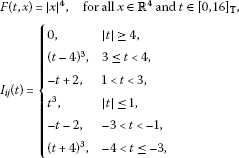for all $i=1,2,3,4$, $j=1,2$. All conditions of Theorem 4.3 hold. According to Theorem 4.3, problem (4.31) has an unbounded sequence of weak solutions.

Remark 4.2 In Theorem 4.3, if we delete the condition ‘$F\left(t,0\right)=0$’, we have the following theorem.

Theorem 4.4 Assume that (A), (F5), (F7), (F8), (F9), (F11) and the following condition are satisfied.

(F13) $F\left(t,x\right)$ is even in x.

Then problem (1.1) has an infinite sequence of distinct weak solutions.

Proof Set $Y={H}^{+}$, $X={H}^{-}\oplus {H}^{0}$ and $E={H}_{T}^{1}$ in Theorem 3.4. Then, from the proof of Theorem 4.3, we know that $E=X\oplus Y$, $dim\left(X\right)<+\mathrm{\infty }$, φ is even, $\phi \in {C}^{1}\left(E,R\right)$ satisfies the (PS) condition, and there are constants ${\rho }_{9},\sigma >0$ such that $\phi {|}_{\partial {B}_{{\rho }_{9}}\cap Y}\ge \sigma$ and $inf\phi \left({B}_{{\rho }_{9}}\cap Y\right)>0$, where $\partial {B}_{{\rho }_{9}}=\left\{u\in E:\parallel u\parallel ={\rho }_{9}\right\}$.

For each finite dimensional subspace $\stackrel{˜}{E}\subset E$, by (4.30), we know that

Consequently, for each finite dimensional subspace ${Y}_{0}\subset Y$, the condition (${\mathrm{\Phi }}_{2}$) holds. Moreover, by $dim\left(X\right)<+\mathrm{\infty }$ and $\phi \in {C}^{1}\left(E,R\right)$, we know that (${\mathrm{\Phi }}_{0}$) holds too. Therefore, the conclusion follows from Theorem 2.6. □

## References

1. Zhou JW, Li YK: Sobolev’s spaces on time scales and its applications to a class of second order Hamiltonian systems on time scales. Nonlinear Anal. 2010, 73: 1375-1388. 10.1016/j.na.2010.04.070

2. Zhou JW, Li YK: Existence of solutions for a class of second-order Hamiltonian systems with impulsive effects. Nonlinear Anal. 2010, 72: 1594-1603. 10.1016/j.na.2009.08.041

3. Nieto JJ, O’Regan D: Variational approach to impulsive differential equations. Nonlinear Anal., Real World Appl. 2009, 10: 680-690. 10.1016/j.nonrwa.2007.10.022

4. Nieto JJ: Impulsive resonance periodic problems of first order. Appl. Math. Lett. 2002, 15: 489-493. 10.1016/S0893-9659(01)00163-X

5. Nieto JJ, Rodriguez-Lopez R: Boundary value problems for a class of impulsive functional equations. Comput. Math. Appl. 2008, 55: 2715-2731. 10.1016/j.camwa.2007.10.019

6. Chen LJ, Chen FD: Dynamic behaviors of the periodic predator-prey system with distributed time delays and impulsive effect. Nonlinear Anal., Real World Appl. 2011, 12: 2467-2473. 10.1016/j.nonrwa.2011.03.002

7. Liu ZS, Chen HB, Zhou TJ: Variational methods to the second-order impulsive differential equation with Dirichlet boundary value problem. Comput. Math. Appl. 2011, 61: 1687-1699. 10.1016/j.camwa.2011.01.042

8. Cichoń M, Satco B, Sikorska-Nowak A: Impulsive nonlocal differential equations through differential equations on time scales. Appl. Math. Comput. 2011, 218: 2449-2458. 10.1016/j.amc.2011.07.057

9. Xiao J, Nieto JJ, Luo ZG: Multiplicity of solutions for nonlinear second order impulsive differential equations with linear derivative dependence via variational methods. Commun. Nonlinear Sci. Numer. Simul. 2012, 17: 426-432. 10.1016/j.cnsns.2011.05.015

10. Carter TE: Necessary and sufficient conditions for optimal impulsive rendezvous with linear equations of motion. Dyn. Control 2000, 10: 219-227. 10.1023/A:1008376427023

11. Pasquero S: On the simultaneous presence of unilateral and kinetic constraints in time-dependent impulsive mechanics. J. Math. Phys. 2006., 47: Article ID 082903

12. Tian Y, Ge WG: Applications of variational methods to boundary value problem for impulsive differential equations. Proc. Edinb. Math. Soc. 2008, 51: 509-527.

13. Aulbach B, Hilger S: Linear dynamic processes with inhomogeneous time scale. Mathematical Research 59. In Nonlinear Dynamics and Quantum Dynamical Systems. Akademie Verlag, Berlin; 1990:9-20.

14. Erbe L, Hilger S: Sturmanian theory on measure chains. Differ. Equ. Dyn. Syst. 1993, 1(3):223-244.

15. Lakshmikantham V, Sivasundaram S, Kaymakcalan B: Dynamic Systems on Measure Chains. Kluwer Academic, Dordrecht; 1996.

16. Agarwal RP, Bohner M: Basic calculus on time scales and some of its applications. Results Math. 1999, 35(1-2):3-22.

17. Agarwal RP, Otero-Espinar V, Perera K, Vivero DR: Basic properties of Sobolev’s spaces on time scales. Adv. Differ. Equ. 2006., 2006: Article ID 38121

18. Bohner M, Peterson A: Dynamic Equations on Time Scales: an Introduction with Applications. Birkhäuser, Boston; 2001.

19. Bohner M, Peterson A: Advances in Dynamic Equations on Time Scales. Birkhäuser, Boston; 2003.

20. Benchohra M, Henderson J, Ntouyas SK, Ouahab A: On first order impulsive dynamic equations on time scales. J. Differ. Equ. Appl. 2004, 6: 541-548.

21. Geng F, Xu Y, Zhu D: Periodic boundary value problems for first-order impulsive dynamic equations on time scales. Nonlinear Anal. 2008, 69: 4074-4087. 10.1016/j.na.2007.10.038

22. Graef JR, Ouahab A: Extremal solutions for nonresonance impulsive functional dynamic equations on time scales. Appl. Math. Comput. 2008, 196: 333-339. 10.1016/j.amc.2007.05.056

23. Wang DB: Positive solutions for nonlinear first-order periodic boundary value problems of impulsive dynamic equations on time scales. Comput. Math. Appl. 2008, 56: 1496-1504. 10.1016/j.camwa.2008.02.038

24. Zhang HT, Li YK: Existence of positive periodic solutions for functional differential equations with impulse effects on time scales. Commun. Nonlinear Sci. Numer. Simul. 2009, 14: 19-26. 10.1016/j.cnsns.2007.08.006

25. Li YK, Zhou JW: Existence of solutions for a class of damped vibration problems on time scales. Adv. Differ. Equ. 2010., 2010: Article ID 727486

26. Li YK, Dong YS: Multiple positive solutions for a fourth-order integral boundary value problem on time scales. Bound. Value Probl. 2011., 2011: Article ID 59

27. Li YK, Shu JY: Multiple positive solutions for first-order impulsive integral boundary value problems on time scales. Bound. Value Probl. 2011., 2011: Article ID 12

28. Li YK, Zhang TW: Multiple positive solutions for second-order -Laplacian dynamic equations with integral boundary conditions. Bound. Value Probl. 2011., 2011: Article ID 867615

29. Su YH, Feng Z: A non-autonomous Hamiltonian system on time scales. Nonlinear Anal. 2012, 75: 4126-4136. 10.1016/j.na.2012.03.003

30. Mawhin J, Willem M: Critical Point Theory and Hamiltonian Systems. Springer, Berlin; 1989.

31. Luan SX, Mao AM: Periodic solutions for a class of non-autonomous Hamiltonian systems. Nonlinear Anal. 2005, 61: 1413-1426. 10.1016/j.na.2005.01.108

32. Rabinowitz PH CBMS Regional Conf. Ser. in Math. 65. In Minimax Methods in Critical Point Theory with Application to Differetial Equations. Am. Math. Soc., Providence; 1986.

33. Bartsch T, Ding YH: Deformation theorems on non-metrizable vector spaces and applications to critical point theory. Math. Nachr. 2006, 279: 1267-1288. 10.1002/mana.200410420

34. Rabinowitz PH: Periodic solutions of Hamiltonian systems. Commun. Pure Appl. Math. 1978, 31: 157-184. 10.1002/cpa.3160310203

35. Wu X, Chen SX, Teng KM: On variational methods for a class of damped vibration problems. Nonlinear Anal. 2008, 68: 1432-1441. 10.1016/j.na.2006.12.043

## Acknowledgements

This work is supported by the National Natural Sciences Foundation of People’s Republic of China under Grant 10971183, the Natural Sciences Foundation of Yunnan Province (2011Y116, 2012FB111, IRTSTYN) and the third batch young skeleton teachers training plan of Yunnan University (XT412003).

## Author information

Authors

### Corresponding author

Correspondence to Yongkun Li.

### Competing interests

The authors declare that they have no competing interests.

### Authors’ contributions

All authors typed, read and approved the final manuscript.

## Rights and permissions

Reprints and Permissions

Zhou, J., Wang, Y. & Li, Y. Existence and multiplicity of solutions for some second-order systems on time scales with impulsive effects. Bound Value Probl 2012, 148 (2012). https://doi.org/10.1186/1687-2770-2012-148

• Accepted:

• Published:

• DOI: https://doi.org/10.1186/1687-2770-2012-148

### Keywords

• nonautonomous second-order systems
• time scales
• impulse
• variational approach